# secml.array¶

## CArray¶

class secml.array.c_array.CArray(data, dtype=None, copy=False, shape=None, tosparse=False)[source]

Creates an array.

Data will be stored in dense form by default.

Parameters
dataarray_like or any built-in datatype

Data to be stored. Can be any array-like structure (sparse or dense) or any built-in list, scalar or string.

dtypestr or dtype, optional

Typecode or data-type to which the array is cast. If None (default), dtype is inferred from input data.

copybool, optional

If False (default) a reference to input data will be stored if possibile. Otherwise, a copy of original data is made first. If data is a nested sequence (a list) or dtype is different, a copy will be made anyway.

shapeint or sequence of ints, optional

Shape of the new array, e.g., ‘(2, 3)’ or ‘2’.

tosparsebool, optional

If True, input data will be converted to sparse format. Otherwise (default), if input is not a CArray, a dense array is returned, or if CArray, its format is preserved.

Examples

>>> from secml.array import CArray

>>> print(CArray([[1, 2], [3, 4]]))
CArray([[1 2]
[3 4]])

>>> print(CArray(True))
CArray([ True])

>>> print(CArray([1,0,3,4], tosparse=True))
CArray(  (0, 0)     1
(0, 2)    3
(0, 3)    4)

>>> print(CArray([1,2,3], dtype=float, shape=(3,1)))  # Custom dtype and shape
CArray([[1.]
[2.]
[3.]])

Attributes
T

Transposed array data.

dtype

Data-type of stored data.

is_vector_like

True if array is vector-like.

isdense

True if data is stored in DENSE form, False otherwise.

issparse

True if data is stored in SPARSE form, False otherwise.

ndim

Number of array dimensions.

nnz

Number of non-zero values in the array.

nnz_data

Return non-zero array elements.

nnz_indices

Index of non-zero array elements.

shape

Shape of stored data, tuple of ints.

size

Size (number of elements) of array.

Methods

 abs(self) Returns array elements without sign. all(self[, axis, keepdims]) Test whether all array elements along a given axis evaluate to True. any(self[, axis, keepdims]) Test whether any array elements along a given axis evaluate to True. append(self, array[, axis]) Append values to the end of an array. apply_along_axis(self, func, axis, \*args, …) Apply function to 1-D slices along the given axis. arange([start, stop, step, dtype, sparse]) Return evenly spaced values within a given interval. argmax(self[, axis]) Indices of the maximum values along an axis. argmin(self[, axis]) Indices of the minimum values along an axis. argsort(self[, axis, kind]) Returns the indices that would sort an array. astype(self, dtype) Copy of the array, casted to a specified type. atleast_2d(self) View original array with at least two dimensions. binary_search(self, value) Returns the index of each input value inside the array. bincount(self[, minlength]) Count the number of occurrences of each value in array of non-negative ints. ceil(self) Return the ceiling of the input, element-wise. clip(self, c_min, c_max) Clip (limit) the values in an array. comblist(list_of_list[, dtype]) Generate a cartesian product of list of list input. concatenate(array1, array2[, axis]) Concatenate a sequence of arrays along the given axis. cos(self) Trigonometric cosine, element-wise. cumsum(self[, axis, dtype]) Return the cumulative sum of the array elements along a given axis. deepcopy(self) Return a deepcopy of current array. diag(self[, k]) Extract a diagonal from array or construct a diagonal array. dot(self, array) Dot product of two arrays. empty(shape[, dtype, sparse]) Return a new array of given shape and type, without filling it. exp(self) Calculate the exponential of all elements in the input array. eye(n_rows[, n_cols, k, dtype, sparse]) Return a 2-D array with ones on the diagonal and zeros elsewhere. find(self, condition) Returns vector-like array elements indices depending on condition. find_2d(self, condition) Returns array elements indices depending on condition. flatten(self) Return a flattened copy of array. floor(self) Return the floor of the input, element-wise. from_iterables(iterables_list) Build an array by chaining elements from objects in the input list. get_data(self) Return stored data as a standard array type. get_nnz(self[, axis]) Counts the number of non-zero values in the array. has_compatible_shape(self, other) Return True if input CArray has a compatible shape. interp(self, x_data, y_data[, return_left, …]) One-dimensional linear interpolation. inv(self) Compute the (multiplicative) inverse of a square matrix. is_inf(self) Test element-wise for positive or negative infinity. is_nan(self) Test element-wise for Not a Number (NaN). is_neginf(self) Test element-wise for negative infinity. is_posinf(self) Test element-wise for positive infinity. item(self) Returns the single element in the array as built-in type. linspace(start, stop[, num, endpoint, sparse]) Return evenly spaced numbers over a specified interval. load(datafile[, dtype, arrayformat, …]) Load array data from plain text file. log(self) Calculate the natural logarithm of all elements in the input array. log10(self) Calculate the base 10 logarithm of all elements in the input array. logical_and(self, array) Element-wise logical AND of array elements. logical_not(self) Element-wise logical NOT of array elements. logical_or(self, array) Element-wise logical OR of array elements. max(self[, axis, keepdims]) Return the maximum of an array or maximum along an axis. maximum(self, array) Element-wise maximum of array elements. mean(self[, axis, dtype, keepdims]) Compute the arithmetic mean along the specified axis. median(self[, axis, keepdims]) Compute the median along the specified axis. meshgrid(xi[, indexing]) Return coordinate matrices from coordinate vectors. min(self[, axis, keepdims]) Return the minimum of an array or minimum along an axis. minimum(self, array) Element-wise minimum of array elements. nan_to_num(self) Replace nan with zero and inf with finite numbers. nanargmax(self[, axis]) Indices of the maximum values along an axis ignoring NaNs. nanargmin(self[, axis]) Indices of the minimum values along an axis ignoring NaNs nanmax(self[, axis, keepdims]) Return the maximum of an array or maximum along an axis ignoring NaNs. nanmin(self[, axis, keepdims]) Return the minimum of an array or minimum along an axis ignoring NaNs. norm(self[, order]) Entrywise vector norm. norm_2d(self[, order, axis, keepdims]) Matrix norm or vector norm along axis. normpdf(self[, mu, sigma]) Return normal distribution function value with mean and standard deviation given for the current array values. ones(shape[, dtype, sparse]) Return a new array of given shape and type, filled with ones. pinv(self[, rcond]) Compute the (Moore-Penrose) pseudo-inverse of a matrix. pow(self, exp) Array elements raised to powers from input exponent, element-wise. prod(self[, axis, dtype, keepdims]) Return the product of array elements over a given axis. rand(shape[, random_state, sparse, density]) Return random floats in the half-open interval [0.0, 1.0). randint(low[, high, shape, random_state, sparse]) Return random integers from low (inclusive) to high (exclusive). randn(shape[, random_state]) Return a sample (or samples) from the “standard normal” distribution. randsample(a[, shape, replace, …]) Generates a random sample from a given array. randuniform([low, high, shape, …]) Return random samples from low (inclusive) to high (exclusive). ravel(self) Return a flattened array. repeat(self, repeats[, axis]) Repeat elements of an array. repmat(self, m, n) Repeat an array M x N times. reshape(self, newshape) Gives a new shape to an array without changing its data. resize(self, newshape[, constant]) Return a new array with the specified shape. rint(self) Round elements of the array to the nearest integer. round(self[, decimals]) Evenly round to the given number of decimals. save(self, datafile[, overwrite]) Save array data into plain text file. sha1(self) Calculate the sha1 hexadecimal hash of array. shuffle(self) Modify array in-place by shuffling its contents. sign(self) Returns element-wise sign of the array. sin(self) Trigonometric sine, element-wise. sort(self[, axis, kind, inplace]) Sort an array. sqrt(self) Compute the positive square-root of an array, element-wise. std(self[, axis, ddof, keepdims]) Compute the standard deviation along the specified axis. sum(self[, axis, keepdims]) Sum of array elements over a given axis. tocsr(self) Return a sparse scipy.sparse.csr_matrix representation of array. todense(self[, dtype, shape]) Converts array to dense format. tolist(self) Return the array as a (possibly nested) list. tondarray(self) Return a dense numpy.ndarray representation of array. tosparse(self[, dtype, shape]) Converts array to sparse format. transpose(self) Returns current array with axes transposed. unique(self[, return_index, return_inverse, …]) Find the unique elements of an array. zeros(shape[, dtype, sparse]) Return a new array of given shape and type, filled with zeros.
property T

Transposed array data.

transpose

bound method to transpose an array.

a
all(self, axis=None, keepdims=True)[source]

Test whether all array elements along a given axis evaluate to True.

Axis selection is available for DENSE format only. For sparse format, logical operation is performed over all the dimensions of the array

Parameters
axisint or None, optional, dense only

Axis or axes along which logical AND between elements is performed. The default (axis = None) is to perform a logical AND over all the dimensions of the input array. If axis is negative, it counts from the last to the first axis.

keepdimsbool, optional, dense only

If this is set to True (default), the result will broadcast correctly against the original array. Otherwise resulting array is flattened.

Returns
bool or CArray

Logical AND element-wise. If axis is None, bool is returned. Otherwise, a CArray of booleans with shape and number of dimensions consistent with the original array and the axis parameter is returned.

Notes

Not a Number (NaN), positive infinity and negative infinity

evaluate to True because these are not equal to zero.

Examples

>>> from secml.array import CArray

>>> print(CArray([[True,False],[True,True]], tosparse=True).all())
False

>>> print(CArray([[True,False],[True,True]]).all(axis=0))
CArray([[ True False]])

>>> print(CArray([-1,0,2,0]).all(axis=0))
CArray([ True False  True False])
>>> print(CArray([-1,0,2,0]).all(axis=1))
CArray([False])

>>> from secml.core.constants import nan, inf
>>> print(CArray([nan, inf, -inf]).all())
True

any(self, axis=None, keepdims=True)[source]

Test whether any array elements along a given axis evaluate to True.

Axis selection is available for DENSE format only. For sparse format, logical operation is performed over all the dimensions of the array

Parameters
axisint or None, optional, dense only

Axis or axes along which logical AND between elements is performed. The default (axis = None) is to perform a logical OR over all the dimensions of the input array. If axis is negative, it counts from the last to the first axis.

keepdimsbool, optional, dense only

If this is set to True (default), the result will broadcast correctly against the original array. Otherwise resulting array is flattened.

Returns
bool or CArray

Logical OR element-wise. If axis is None, bool is returned. Otherwise, a CArray of booleans with shape and number of dimensions consistent with the original array and the axis parameter is returned.

Notes

Not a Number (NaN), positive infinity and negative infinity

evaluate to True because these are not equal to zero.

Examples

>>> from secml.array import CArray

>>> print(CArray([[True,False],[True,True]], tosparse=True).any())
True

>>> print(CArray([[True,False],[True,False]]).any(axis=0))
CArray([[ True False]])

>>> print(CArray([-1,0,2,0]).any(axis=0))
CArray([ True False  True False])
>>> print(CArray([-1,0,2,0]).any(axis=1))
CArray([ True])

>>> from secml.core.constants import nan, inf
>>> print(CArray([nan, inf, -inf]).any())
True

append(self, array, axis=None)[source]

Append values to the end of an array.

Parameters
arrayCArray or array_like

Second array.

axisint or None, optional

The axis along which values are appended. If axis is None, both arrays are flattened before use.

Returns
CArray

A copy of array with values appended to axis. Note that append does not occur in-place: a new array is allocated and filled. If axis is None, out is a flattened array. Always return an array with the same format of the first array.

Notes

Differently from numpy, we manage flat vectors as 2-Dimensional of shape (1, array.size). Consequently, result of appending a flat array to a flat array is 1-Dimensional only if axis=1. Appending a flat array to a 2-Dimensional array, or vice versa, always results in a 2-Dimensional array.

Examples

>>> from secml.array import CArray

>>> print(CArray([[1,2],[3,4]]).append([,]))
CArray([ 1  2  3  4 11 22])
>>> print(CArray([[1,2],[3,4]]).append([[11,22]], axis=0))
CArray([[ 1  2]
[ 3  4]
[11 22]])

>>> print(CArray([[1,2],[3,4]]).append(CArray([,], tosparse=True)))
CArray([ 1  2  3  4 11 22])
>>> array = CArray([[1,2],[3,4]], tosparse=True).append([,])
>>> print(array)
CArray(  (0, 0) 1
(0, 1)        2
(0, 2)        3
(0, 3)        4
(0, 4)        11
(0, 5)        22)

>>> print(CArray([1,2]).append([11,22]))
CArray([ 1  2 11 22])

>>> print(CArray([1,2]).append([11,22], axis=0))
CArray([[ 1  2]
[11 22]])
>>> print(CArray([1,2]).append([11,22], axis=1))
CArray([ 1  2 11 22])

apply_along_axis(self, func, axis, *args, **kwargs)[source]

Apply function to 1-D slices along the given axis.

func should accept 1-D arrays and return a single scalar or a 1-D array.

Only 1-D and 2-D arrays are currently supported.

Parameters
funcfunction

Function object to apply along the given axis. Must return a single scalar or a 1-D array.

axisint

Axis along which to apply the function.

*args, **kwargsoptional

Any other input value for func.

Returns
CArray

1-Dimensional array of size data.shape with the output of func for each row in data. Datatype of output array is always float.

Examples

>>> from secml.array import CArray

>>> a = CArray([[1,2],[10,20],[100,200]])

>>> def return_sum(x):
...     return x.sum()

>>> print(a.apply_along_axis(return_sum, axis=0))  # Column-wise
CArray([111. 222.])

>>> print(a.apply_along_axis(return_sum, axis=1))  # Row-wise
CArray([  3.  30. 300.])

classmethod arange(start=None, stop=None, step=1, dtype=None, sparse=False)[source]

Return evenly spaced values within a given interval.

Values are generated within the half-open interval [start, stop). For integer arguments the function is equivalent to the Python built-in range function, but returns an ndarray rather than a list.

When using a non-integer step, such as 0.1, the results will often not be consistent. It is better to use linspace for these cases.

Parameters
startscalar, optional

Start of interval. The interval includes this value. The default start value is 0.

stopscalar

End of interval. The interval does not include this value, except in some cases where step is not an integer and floating point round-off affects the length of the output.

stepscalar, optional

Spacing between values. For any output out, this is the distance between two adjacent values, out[i+1] - out[i]. The default step size is 1. If step is specified, start must also be given.

dtypestr or dtype, optional

The type of the output array. If dtype is not given, infer the data type from the other input arguments.

sparsebool, optional

If False (default) a dense array will be returned. Otherwise, a sparse array is created.

Returns
CArray

Array of evenly spaced values. For floating point arguments, the length of the result is ceil((stop - start)/step). Because of floating point overflow, this rule may result in the last element of out being greater than stop.

Warning

When sparse is True, array is created as dense and then converted to sparse format. Consequently, the performance of this method is not comparable to other sparse array creation routines.

CArray.linspace

Evenly spaced numbers with handling of endpoints.

Examples

>>> from secml.array import CArray

>>> print(CArray.arange(4))
CArray([0 1 2 3])

>>> print(CArray.arange(4.0))
CArray([0. 1. 2. 3.])

>>> print(CArray.arange(4.0, dtype=int))
CArray([0 1 2 3])

>>> print(CArray.arange(0, 4))
CArray([0 1 2 3])

>>> print(CArray.arange(0, 4, 0.8))
CArray([0.  0.8 1.6 2.4 3.2])

argmax(self, axis=None)[source]

Indices of the maximum values along an axis.

Parameters
axisint, None, optional

If None (default), array is flattened before computing index, otherwise the specified axis is used.

Returns
int or CArray

Index of the maximum of the array. If axis is None, int is returned. Otherwise, a CArray with shape and number of dimensions consistent with the original array and the axis parameter is returned.

Notes

In case of multiple occurrences of the maximum values, the

indices corresponding to the first occurrence are returned.

Examples

>>> from secml.array import CArray

>>> print(CArray([-1, 0, 3]).argmax())
2

>>> print(CArray([[-1, 0],[4, 3]]).argmax(axis=0))  # We return the index of maximum for each column
CArray([[1 1]])

>>> print(CArray([[-1, 0],[4, 3]]).argmax(axis=1))  # We return the index of maximum for each row
CArray([
])

>>> print(CArray([-3,0,1,2]).argmax(axis=0))
CArray([0 0 0 0])
>>> print(CArray([-3,0,1,2]).argmax(axis=1))
CArray()

argmin(self, axis=None)[source]

Indices of the minimum values along an axis.

Parameters
axisint, None, optional

If None (default), array is flattened before computing index, otherwise the specified axis is used.

Returns
int or CArray

Index of the minimum of the array. If axis is None, int is returned. Otherwise, a CArray with shape and number of dimensions consistent with the original array and the axis parameter is returned.

Notes

In case of multiple occurrences of the minimum values, the

indices corresponding to the first occurrence are returned.

Examples

>>> from secml.array import CArray

>>> print(CArray([-1, 0, 3]).argmin())
0

>>> print(CArray([[-1, 0],[4, 3]]).argmin(axis=0))  # We return the index of minimum for each column
CArray([[0 0]])

>>> print(CArray([[-1, 0],[4, 3]]).argmin(axis=1))  # We return the index of maximum for each row
CArray([
])

>>> print(CArray([-3,0,1,2]).argmin(axis=0))
CArray([0 0 0 0])
>>> print(CArray([-3,0,1,2]).argmin(axis=1))
CArray()

argsort(self, axis=-1, kind='quicksort')[source]

Returns the indices that would sort an array.

Perform an indirect sort along the given axis using the algorithm specified by the kind keyword. It returns an array of indices of the same shape as a that index data along the given axis in sorted order.

Parameters
axisint or None, optional

Axis along which to sort. The default is -1 (the last axis). If None, the flattened array is used.

kind{‘quicksort’, ‘mergesort’, ‘heapsort’}, optional

Sorting algorithm to use. Default ‘quicksort’. For sparse arrays, only ‘quicksort’ is available.

Returns
CArray

Array of indices that sort the array along the specified axis. In other words, array[index_array] yields a sorted array.

numpy.sort

Description of different sorting algorithms.

CArray.sort

In-Place sorting of array.

Examples

>>> from secml.array import CArray

>>> print(CArray([0,-3,5]).argsort())
CArray([1 0 2])

>>> print(CArray([[0,-3],[5,1]]).argsort(axis=1))  # Sorting of each row
CArray([[1 0]
[1 0]])

>>> print(CArray([[0,-3],[5,1]]).argsort(axis=None))  # Sorting the flattened array
CArray([1 0 3 2])

astype(self, dtype)[source]

Copy of the array, casted to a specified type.

Parameters
dtypestr or dtype

Typecode or data-type to which the array is cast.

Returns
CArray

Copy of the original array casted to new data type.

Examples

>>> from secml.array import CArray

>>> print(CArray([1, 2, 3]).astype(float))
CArray([1. 2. 3.])

>>> print(CArray([1.1, 2.1, 3.1], tosparse=True).astype(int))
CArray(  (0, 0) 1
(0, 1)        2
(0, 2)        3)

atleast_2d(self)[source]

View original array with at least two dimensions.

A copy is made only if needed.

Returns
outCArray

Array with array.ndim >= 2.

Notes

Sparse arrays are always 2 dimensional so this method returns a view (if possible) of the original array without any changes.

Examples

>>> from secml.array import CArray

>>> print(CArray([1,2,3]).atleast_2d())
CArray([[1 2 3]])


Returns the index of each input value inside the array.

DENSE ARRAYS ONLY

If value is not found inside the array, the index of the closest value will be returned. Array will be flattened before search.

Parameters
valuescalar or CArray

Element or array of elements to search inside the flattened array.

Returns
int or CArray

Position of input value, or the closest one, inside flattened array. If value is an array, a CArray with the position of each value element is returned.

Examples

>>> from secml.array import CArray

>>> print(CArray([[0,0.1],[0.4,1.0]]).binary_search(0.3))
2

>>> print(CArray([1,2,3,4]).binary_search(10))
3

>>> print(CArray([1,2,3,4]).binary_search(CArray([-10,1,2.2,10])))
CArray([0 0 1 3])

bincount(self, minlength=0)[source]

Count the number of occurrences of each value in array of non-negative ints.

Only vector like arrays of integer dtype are supported.

Parameters
minlengthint, optional

A minimum number of bins for the output.

Returns
CArray

The occurrence number for every different element of array. The length of output array is equal to a.max()+1 if an argument for the parameter minlenght is not provided.

Examples

>>> from secml.array import CArray

>>> a = CArray([1, 2, 3, 1, 6], tosparse=True)
>>> print(a.bincount())
CArray([0 2 1 1 0 0 1])

ceil(self)[source]

Return the ceiling of the input, element-wise.

The ceil of the scalar x is the smallest integer i, such that i >= x.

Returns
out_ceilCArray

The ceiling of each element in x, with float dtype.

round

Evenly round to the given number of decimals.

floor

Return the floor of the input, element-wise.

Examples

>>> from secml.array import CArray

>>> print(CArray([-1.7, -1.5, -0.2, 0.2, 1.5, 1.7, 2.0]).ceil())
CArray([-1. -1. -0.  1.  2.  2.  2.])

>>> # Array with dtype == int is upcasted to float before ceiling
>>> print(CArray([[-2, -1], [1, 1]], tosparse=True).ceil())
CArray(  (0, 0) -2.0
(0, 1)        -1.0
(1, 0)        1.0
(1, 1)        1.0)

clip(self, c_min, c_max)[source]

Clip (limit) the values in an array.

DENSE FORMAT ONLY

Given an interval, values outside the interval are clipped to the interval edges. For example, if an interval of [0, 1] is specified, values smaller than 0 become 0, and values larger than 1 become 1.

Parameters
c_min, c_maxint

Clipping intervals.

Returns
CArray

Returns a new array containing the clipped array elements. Dtype of the output array depends on the dtype of original array and on the dtype of the clipping limits.

Examples

>>> from secml.array import CArray

>>> print(CArray([[1,2],[3,4]]).clip(2, 4))
CArray([[2 2]
[3 4]])

>>> from secml.core.constants import inf

>>> # inf is a float, array will be casted accordingly
>>> print(CArray([[1,2],[3,4]]).clip(-inf, 2))
CArray([[1. 2.]
[2. 2.]])

classmethod comblist(list_of_list, dtype=<class 'float'>)[source]

Generate a cartesian product of list of list input.

Parameters
list_of_listlist of list

1-D arrays to form the cartesian product of.

dtypestr or dtype

Datatype of output array. Default float.

Returns
CArray

2-D array of shape (M, len(arrays)) containing cartesian products between input arrays.

Examples

>>> print(CArray.comblist([[1, 2, 3], [4, 5], [6, 7]]))
CArray([[1. 4. 6.]
[1. 4. 7.]
[1. 5. 6.]
[1. 5. 7.]
[2. 4. 6.]
[2. 4. 7.]
[2. 5. 6.]
[2. 5. 7.]
[3. 4. 6.]
[3. 4. 7.]
[3. 5. 6.]
[3. 5. 7.]])

>>> print(CArray.comblist([[1, 2], ], dtype=int))
CArray([[1 3]
[2 3]])

classmethod concatenate(array1, array2, axis=1)[source]

Concatenate a sequence of arrays along the given axis.

The arrays must have the same shape, except in the dimension corresponding to axis (the second, by default).

This function preserves input masks if available.

Parameters
array1CArray or array_like

First array. If array1 is not an array, a CArray will be created before concatenating.

array2CArray or array_like

Second array. If array2 is not an array, a CArray will be created before concatenating.

axisint or None, optional

The axis along which the arrays will be joined. Default is 1. If None, both arrays are ravelled before concatenation.

Returns
CArray

The concatenated array. If first array is sparse, return sparse.

Notes

Differently from numpy, we manage flat vectors as 2-Dimensional of shape (1, array.size). Consequently, concatenation result of 2 flat arrays is a flat array only when axis=1.

Examples

>>> from secml.array import CArray

>>> print(CArray.concatenate([[1,2],[3,4]], [,]))
CArray([[ 1  2 11]
[ 3  4 22]])
>>> print(CArray.concatenate([[1,2],[3,4]], [[11,22]], axis=0))
CArray([[ 1  2]
[ 3  4]
[11 22]])

>>> print(CArray.concatenate([[1,2],[3,4]], CArray([,], tosparse=True)))
CArray([[ 1  2 11]
[ 3  4 22]])
>>> array = CArray.concatenate(CArray([[1,2],[3,4]], tosparse=True), [,])
>>> print(array)
CArray(  (0, 0) 1
(0, 1)        2
(0, 2)        11
(1, 0)        3
(1, 1)        4
(1, 2)        22)

>>> print(CArray.concatenate([1,2], [11,22]))
CArray([ 1  2 11 22])

>>> print(CArray.concatenate([1,2], [11,22], axis=0))
CArray([[ 1  2]
[11 22]])
>>> print(CArray.concatenate([1,2], [11,22], axis=1))
CArray([ 1  2 11 22])

cos(self)[source]

Trigonometric cosine, element-wise.

DENSE FORMAT ONLY

The array elements are considered angles, in radians (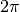rad equals 360 degrees).

Returns
CArray

New array with trigonometric cosine element-wise.

Examples

>>> from secml.array import CArray
>>> from secml.core.constants import pi

>>> print((CArray([0,90,180,270,360,-90,-180,-270])*pi/180).cos().round())
CArray([ 1.  0. -1. -0.  1.  0. -1. -0.])

>>> print((CArray([[45,135],[225,315]])*pi/180).cos())
CArray([[ 0.707107 -0.707107]
[-0.707107  0.707107]])

cumsum(self, axis=None, dtype=None)[source]

Return the cumulative sum of the array elements along a given axis.

DENSE FORMAT ONLY

Parameters
axisint or None, optional

Axis along which the cumulative sum is computed. The default (None) is to compute the cumsum over the flattened array.

dtypedtype or None, optional

Type of the returned array and of the accumulator in which the elements are summed. If dtype is not specified, it defaults to the dtype of a, unless a has an integer dtype with a precision less than that of the default platform integer. In that case, the default platform integer is used.

Returns
CArray

New array with cumulative sum of elements. If axis is None, flat array with same size of input array. If axis is not None, same shape of input array.

Examples

>>> from secml.array import CArray

>>> print(CArray([-3,0,2]).cumsum())
CArray([-3 -3 -1])

>>> print(CArray([-3,0,1,2]).cumsum(axis=0))
CArray([-3  0  1  2])
>>> print(CArray([-3,0,1,2]).cumsum(axis=1))
CArray([-3 -3 -2  0])

>>> print(CArray([[-3,0],[1,2]]).cumsum(dtype=float))
CArray([-3. -3. -2.  0.])

>>> print(CArray([[-3,0],[1,2]]).cumsum(axis=1))
CArray([[-3 -3]
[ 1  3]])

d
diag(self, k=0)[source]

Extract a diagonal from array or construct a diagonal array.

Parameters
kint, optional

Diagonal index. Default is 0. Use k > 0 for diagonals above the main diagonal, k < 0 for diagonals below the main diagonal.

Returns
CArray

The extracted diagonal or constructed diagonal dense array. If array is 2-Dimensional, returns its k-th diagonal. Depending on numpy version resulting array can be read-only or a view of the original array’s diagonal. To make output array writable, use deepcopy(). If array is vector-like, return a 2-D array with the array on the k-th diagonal.

Examples

>>> from secml.array import CArray

>>> print(CArray([[1, 2, 3], [10, 20, 30]]).diag(k=1))
CArray([ 2 30])

>>> print(CArray([[2, 1]], tosparse=True).diag())
CArray(  (0, 0) 2
(1, 1)        1)

>>> print(CArray([1, 2, 3]).diag(k=1))
CArray([[0 1 0 0]
[0 0 2 0]
[0 0 0 3]
[0 0 0 0]])

dot(self, array)[source]

Dot product of two arrays.

For 2-D arrays it is equivalent to matrix multiplication. If both arrays are dense flat (rows), it is equivalent to the inner product of vectors (without complex conjugation).

Format of output array is the same of the first product argument.

Parameters
arrayCArray

Second argument of dot product.

Returns
scalar or CArray

Result of dot product. A CArray with the same format of first argument or scalar if out.size == 1.

Examples

>>> from secml.array import CArray

>>> print(CArray([[1,1],[2,2]]).dot(CArray([[1,1],[0,0]], tosparse=True)))
CArray([[1 1]
[2 2]])

>>> print(CArray([10,20]).dot(CArray([,], tosparse=True)))
10


OUTER PRODUCT

>>> print(CArray([,]).dot(CArray([1,0], tosparse=True)))
CArray([[10  0]
[20  0]])


INNER PRODUCT BETWEEN VECTORS

>>> print(CArray([10,20]).dot(CArray([1,0])))
10


Inner product between vector-like arrays is a matrix multiplication

>>> print(CArray([10,20]).dot(CArray([1,0], tosparse=True).T))
10
>>> print(CArray([10,20], tosparse=True).dot(CArray([1,0]).T))
10

property dtype

Data-type of stored data.

classmethod empty(shape, dtype=<class 'float'>, sparse=False)[source]

Return a new array of given shape and type, without filling it.

Parameters
shapeint or tuple

Shape of the new array, e.g., 2 or (2,3).

dtypestr or dtype, optional

The desired data-type for the array. Default is float.

sparsebool, optional

If False (default) a dense array will be returned. Otherwise, a sparse array is returned.

Returns
CArray

Array of arbitrary values with the given properties.

Notes

.empty, unlike .zeros, does not set the array values to zero, and may therefore be marginally faster. On the other hand, it requires the user to manually set all the values in the array, and should be used with caution.

Examples

>>> from secml.array import CArray

>>> array = CArray.empty(3)
>>> print(array
CArray([  0.00000000e+000   4.94944794e+173   6.93660640e-310])  # random

>>> array = CArray.empty((2,1), dtype=int, sparse=True)
>>> print(array)
CArray()
>>> print(array.shape)
(2, 1)

exp(self)[source]

Calculate the exponential of all elements in the input array.

DENSE FORMAT ONLY

Returns
CArray

New array with element-wise exponential of current data.

Notes

The irrational number e is also known as Euler’s number. It is approximately 2.718281, and is the base of the natural logarithm, ln (this means that, if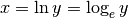, then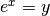. For real input, exp(x) is always positive.

For complex arguments, x = a + ib, we can write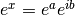. The first term,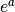, is already known (it is the real argument, described above). The second term,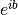, is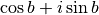, a function with magnitude 1 and a periodic phase.

Examples

>>> from secml.array import CArray

>>> print(CArray([0,1,3]).exp())
CArray([ 1.        2.718282 20.085537])

classmethod eye(n_rows, n_cols=None, k=0, dtype=<class 'float'>, sparse=False)[source]

Return a 2-D array with ones on the diagonal and zeros elsewhere.

Parameters
n_rowsint

Number of rows in the output.

n_colsint or None, optional

Number of columns in the output. If None, defaults to n_rows.

kint, optional

Index of the diagonal: 0 (the default) refers to the main diagonal, a positive value refers to an upper diagonal, and a negative value to a lower diagonal.

dtypestr or dtype, optional

Data-type of the returned array.

sparsebool, optional

If False (default) a dense array will be returned. Otherwise, a sparse array will be created.

Returns
CArray

An array where all elements are equal to zero, except for the k-th diagonal, whose values are equal to one.

Examples

>>> from secml.array import CArray
>>> array = CArray.eye(2)
>>> print(array)
CArray([[1. 0.]
[0. 1.]])

>>> array = CArray.eye(2, 3, k=1, dtype=int, sparse=True)
>>> print(array)
CArray(  (0, 1) 1
(1, 2)        1)

>>> print(array.shape)
(2, 3)

find(self, condition)[source]

Returns vector-like array elements indices depending on condition.

Parameters
conditionCArray

Array with booleans representing desired condition.

Returns
list

List with indices corresponding to array elements where condition is True.

find_2d

find method for arrays of generic shape.

Examples

>>> from secml.array import CArray

>>> array = CArray([1,0,-6,2,0])
>>> array_find = array.find(array > 0)
>>> print(array_find)
[0, 3]
>>> print(array[array_find])
CArray([1 2])

>>> array = CArray([[1,0,-6,2,0]])
>>> array_find = array.find(array == 0)
>>> print(array_find)
[1, 4]
>>> print(array[array_find].shape)
(1, 2)

>>> array = CArray([[1,0,-6,2,0]], tosparse=True)
>>> array_find = array.find(array == 0)
>>> print(array_find)
[1, 4]
>>> print(array[array_find].shape)
(1, 2)

find_2d(self, condition)[source]

Returns array elements indices depending on condition.

Parameters
conditionCArray

Array with booleans representing desired condition.

Returns
list

List of len(out_find) == ndim with indices corresponding to array elements where condition is True. Es. for matrices, out_find holds the indices of rows, out_find the indices of columns.

Notes

Using .find_2d() output for indexing original array always result in a ravelled array with elements which corresponding condition was True.

Examples

>>> from secml.array import CArray

>>> array = CArray([[1,0],[-6,3],[2,7]])
>>> array_find = array.find_2d(array > 0)
>>> print(array_find)
[[0, 1, 2, 2], [0, 1, 0, 1]]
>>> print(array[array_find])
CArray([1 3 2 7])

>>> array = CArray([[1,0],[-6,0],[2,0]], tosparse=True)
>>> array_find = array.find_2d(array == 0)
>>> print(array_find)
[[0, 1, 2], [1, 1, 1]]
>>> print(array[array_find].shape)
(1, 3)

>>> array = CArray([1,0,2])
>>> array_find = array.find_2d(array > 0)
>>> print(array_find)
[[0, 0], [0, 2]]
>>> print(array[array_find])
CArray([1 2])

flatten(self)[source]

Return a flattened copy of array.

For dense format a 1-dim array, containing the elements of the input, is returned. For sparse format a (1 x array.size) array will be returned.

Returns
CArray

Output of the same dtype as a, of shape (array.size,) for dense format or (1,array.size) for sparse format.

Examples

>>> from secml.array import CArray

>>> print(CArray([[1,2],[3,4]]).flatten())
CArray([1 2 3 4])

>>> print(CArray([,,], tosparse=True).flatten())
CArray(  (0, 0) 1
(0, 1)        2
(0, 2)        3)

floor(self)[source]

Return the floor of the input, element-wise.

The floor of the scalar x is the largest integer i, such that i <= x.

Returns
out_floorCArray

The floor of each element in x, with float dtype.

CArray.round

Evenly round to the given number of decimals.

CArray.ceil

Return the ceiling of the input, element-wise.

Notes

Some spreadsheet programs calculate the “floor-towards-zero”, in other words floor(-2.5) == -2. We instead uses the definition of floor where floor(-2.5) == -3.

Examples

>>> from secml.array import CArray

>>> print(CArray([-1.7, -1.5, -0.2, 0.2, 1.5, 1.7, 2.0]).floor())
CArray([-2. -2. -1.  0.  1.  1.  2.])

>>> # Array with dtype == int is upcasted to float before flooring
>>> print(CArray([[-2, -1], [1, 1]], tosparse=True).floor())
CArray(  (0, 0) -2.0
(0, 1)        -1.0
(1, 0)        1.0
(1, 1)        1.0)

classmethod from_iterables(iterables_list)[source]

Build an array by chaining elements from objects in the input list.

Parameters
iterables_listlist of iterable

List of iterables to chain. Valid objects are CArrays, lists, tuples, and any other iterable. N-Dimensional arrays are flattened before chaining.

Returns
CArray

Flat CArray with all values chained from input objects.

Examples

>>> from secml.array import CArray

>>> print(CArray.from_iterables([[1, 2], (3, 4), CArray([5, 6])]))
CArray([1 2 3 4 5 6])

>>> print(CArray.from_iterables([CArray([1, 2]), CArray([[3, 4], [5, 6]])]))
CArray([1 2 3 4 5 6])

get_data(self)[source]

Return stored data as a standard array type.

Returns
np.ndarray or scipy.sparse.csr_matrix

If array is dense, a np.ndarray is returned. If array is sparse, a scipy.sparse.csr_matrix is returned.

tondarray

returns a np.ndarray, regardless of array format.

tocsr

returns a scipy.sparse.csr_matrix, regardless of array format.

get_nnz(self, axis=None)[source]

Counts the number of non-zero values in the array.

Parameters
axisbool or None, optional

Axis or tuple of axes along which to count non-zeros. Default is None, meaning that non-zeros will be counted along a flattened version of the array.

Returns
countCArray or int

Number of non-zero values in the array along a given axis. Otherwise, the total number of non-zero values in the array is returned.

Examples

>>> from secml.array import CArray

>>> a = CArray([[1,2],[0,5],[0,0],[2,0]])
>>> print(a.get_nnz())  # Total number of non-zero elements
4
>>> print(a.get_nnz(axis=0))  # Number of non-zero elements for each column
CArray([2 2])
>>> print(a.get_nnz(axis=1))  # Number of non-zero elements for each row
CArray([2 1 0 1])

has_compatible_shape(self, other)[source]

Return True if input CArray has a compatible shape.

Two CArrays can be considered compatible if both have the same shape or both are vector-like.

Parameters
otherCArray

Array to check for shape compatibility

Returns
bool

True if input array has compatible shape with current array.

is_vector_like

check if an array is vector-like.

Examples

>>> from secml.array import CArray

>>> print(CArray([[1,2]]).has_compatible_shape(CArray([,])))
False

>>> print(CArray([1,2]).has_compatible_shape(CArray([1,2,3])))
False

>>> print(CArray([[1,2]], tosparse=True).has_compatible_shape(CArray([1,2])))
True

interp(self, x_data, y_data, return_left=None, return_right=None)[source]

One-dimensional linear interpolation.

DENSE FORMAT ONLY

Returns the 1-D piecewise linear interpolant to a function with given values at discrete data-points.

Parameters
x_dataCArray

Flat array of floats with the x-coordinates of the data points, must be increasing.

y_dataCArray

Flat array of floats with the y-coordinates of the data points, same length as x_data.

return_leftfloat, optional

Value to return for x < x_data, default is y_data.

return_rightfloat, optional

Value to return for x > x_data[-1], default is y_data[-1].

Returns
CArray

The interpolated values, same shape as x.

Notes

The function does not check that the x-coordinate sequence x_data is increasing. If x_data is not increasing, the results are nonsense.

Examples

>>> from secml.array import CArray
>>> from secml.figure import CFigure
>>> from secml.core.constants import pi

>>> fig = CFigure(fontsize=14)
>>> x_array = CArray.linspace(0, 2*pi, 10)
>>> y_array = x_array.sin()
>>> x_vals = CArray.linspace(0, 2*pi, 50)

>>> y_interp = x_vals.interp(x_array, y_array)

>>> fig.sp.plot(x_array, y_array, 'o')
>>> fig.sp.plot(x_vals, y_interp, '-xr')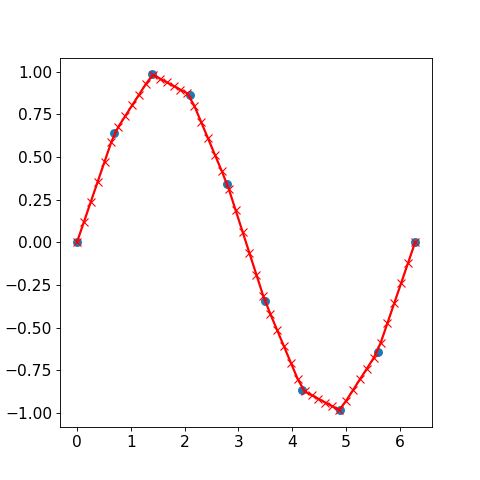inv(self)[source]

Compute the (multiplicative) inverse of a square matrix.

Given a square matrix a, return the matrix inv satisfying dot(array, array_inv) = dot(array_inv, array) = eye(array.shape).

Returns
array_invCArray

(Multiplicative) inverse of the square matrix.

Raises
LinAlgErrordense only

If array is not square or inversion fails.

ValueErrorsparse only

If array is not square or inversion fails

Notes

If the inverse of a sparse array is expected to be non-sparse, it will likely be faster to convert array to dense first.

Examples

>>> from secml.array import CArray

>>> array = CArray([[1., 2.], [3., 4.]])
>>> array_inv = array.inv()
>>> (array.dot(array_inv).round() == CArray.eye(2)).all()
True
>>> (array_inv.dot(array).round() == CArray.eye(2)).all()
True

>>> print(CArray([[1., 2.], [3., 4.]], tosparse=True).inv().round(1))
CArray(  (0, 0) -2.0
(0, 1)        1.0
(1, 0)        1.5
(1, 1)        -0.5)

>>> CArray([[1.,2.,3.], [4., 5.,6.]]).inv()
Traceback (most recent call last):
...
numpy.linalg.linalg.LinAlgError: Last 2 dimensions of the array must be square

is_inf(self)[source]

Test element-wise for positive or negative infinity.

Returns
CArray

Array of the same shape as x, with True where x == +/-inf, otherwise False.

Examples

>>> from secml.core.constants import inf, nan
>>> from secml.array import CArray

>>> a = CArray([1, inf, -inf, nan, 4.5])
>>> print(a.is_inf())
CArray([False  True  True False False])

is_nan(self)[source]

Test element-wise for Not a Number (NaN).

Returns
CArray

Array of the same shape as x, with True where x == nan, otherwise False.

Examples

>>> from secml.core.constants import inf, nan
>>> from secml.array import CArray

>>> a = CArray([1, inf, -inf, nan, 4.5])
>>> print(a.is_nan())
CArray([False False False  True False])

is_neginf(self)[source]

Test element-wise for negative infinity.

Returns
CArray

Array of the same shape as x, with True where x == -inf, otherwise False.

Examples

>>> from secml.core.constants import inf, nan
>>> from secml.array import CArray

>>> a = CArray([1, inf, -inf, nan, 4.5])
>>> print(a.is_neginf())
CArray([False False  True False False])

is_posinf(self)[source]

Test element-wise for positive infinity.

Returns
CArray

Array of the same shape as x, with True where x == +inf, otherwise False.

Examples

>>> from secml.core.constants import inf, nan
>>> from secml.array import CArray

>>> a = CArray([1, inf, -inf, nan, 4.5])
>>> print(a.is_posinf())
CArray([False  True False False False])

property is_vector_like

True if array is vector-like.

An array is vector-like when 1-Dimensional or 2-Dimensional with shape == 1.

Returns
bool

True if array is vector-like.

Examples

>>> from secml.array import CArray

>>> a = CArray([1,2,3])
>>> a.is_vector_like
True

>>> a = CArray([1,2,3], tosparse=True)  # sparse arrays always 2-D
>>> a.is_vector_like
True

>>> a = CArray([[1,2],[3,4]])
>>> a.is_vector_like
False

property isdense

True if data is stored in DENSE form, False otherwise.

Returns
bool

True if data is stored in DENSE form, False otherwise.

property issparse

True if data is stored in SPARSE form, False otherwise.

Returns
bool

True if data is stored in SPARSE form, False otherwise.

item(self)[source]

Returns the single element in the array as built-in type.

Returns
int, float, bool, str

The single element in the array.

Examples

>>> from secml.array import CArray

>>> print(CArray().item())
1

>>> print(CArray([[1.]]).item())
1.0

>>> print(CArray(, tosparse=True).item())
1

>>> print(CArray([1,2,3]).item())
Traceback (most recent call last):
...
ValueError: cannot use .item(). Array has size 3

>>> print(CArray([]).item())
Traceback (most recent call last):
...
ValueError: cannot use .item(). Array has size 0

classmethod linspace(start, stop, num=50, endpoint=True, sparse=False)[source]

Return evenly spaced numbers over a specified interval.

Returns num evenly spaced float samples, calculated over the interval [start, stop ]. The endpoint of the interval can optionally be excluded.

Parameters
startscalar

The starting value of the sequence.

stopscalar

The end value of the sequence, unless endpoint is set to False. In that case, the sequence consists of all but the last of num + 1 evenly spaced samples, so that stop is excluded. Note that the step size changes when endpoint is False.

numint, optional

Number of samples to generate. Default is 50.

endpointbool, optional

If True, stop is the last sample. Otherwise, it is not included. Default is True.

sparsebool, optional

If False (default) a dense array will be returned. Otherwise, a sparse array is created.

Returns
CArray

There are num equally spaced samples in the closed interval [start, stop] or the half-open interval [start, stop) (depending on whether endpoint is True or False).

Warning

When sparse is True, array is created as dense and then converted to sparse format. Consequently, the performance of this method is not comparable to other sparse array creation routines.

CArray.arange

Similar to linspace, but uses a specific step size.

Examples

>>> from secml.array import CArray

>>> array = CArray.linspace(3.0, 4, num=5)
>>> print(array)
CArray([3.   3.25 3.5  3.75 4.  ])

>>> array = CArray.linspace(3, 4., num=5, endpoint=False)
>>> print(array)
CArray([3.  3.2 3.4 3.6 3.8])

classmethod load(datafile, dtype=<class 'float'>, arrayformat='dense', startrow=0, skipend=0, cols=None)[source]

Load array data from plain text file.

The default encoding is utf-8.

Parameters
datafilestr or file_handle

File or filename to read. If the filename extension is gz or bz2, the file is first decompressed.

dtypestr, dtype, optional

Data type of the resulting array, default ‘float’. If None, the dtype will be determined by the contents of the file.

arrayformat{‘dense’, ‘sparse’}, optional

Format of array to load, default ‘dense’.

startrowint, optional, dense only

skipendint, optional, dense only

Number of lines to skip from the end of the file when reading.

cols{CArray, int, tuple}, optional, dense only

Columns to load from target file.

Returns
CArray

log(self)[source]

Calculate the natural logarithm of all elements in the input array.

DENSE FORMAT ONLY

Returns
CArray

New array with element-wise natural logarithm of current data.

Notes

Logarithm is a multivalued function: for each x there is an infinite number of z such that exp(z) = x. The convention is to return the z whose imaginary part lies in [-pi, pi].

For real-valued input data types, log always returns real output. For each value that cannot be expressed as a real number or infinity, it yields nan and sets the invalid floating point error flag.

For complex-valued input, log is a complex analytical function that has a branch cut [-inf, 0] and is continuous from above on it. log handles the floating-point negative zero as an infinitesimal negative number, conforming to the C99 standard.

References

1

M. Abramowitz and I.A. Stegun, “Handbook of Mathematical Functions”, 10th printing, 1964, pp. 67. http://www.math.sfu.ca/~cbm/aands/

2

Wikipedia, “Logarithm”. http://en.wikipedia.org/wiki/Logarithm

Examples

>>> from secml.array import CArray

>>> print(CArray([0,1,3]).log())
CArray([    -inf 0.       1.098612])

log10(self)[source]

Calculate the base 10 logarithm of all elements in the input array.

DENSE FORMAT ONLY

Returns
CArray

New array with element-wise base 10 logarithm of current data.

Notes

Logarithm is a multivalued function: for each x there is an infinite number of z such that 10**z = x. The convention is to return the z whose imaginary part lies in [-pi, pi].

For real-valued input data types, log10 always returns real output. For each value that cannot be expressed as a real number or infinity, it yields nan and sets the invalid floating point error flag.

For complex-valued input, log10 is a complex analytical function that has a branch cut [-inf, 0] and is continuous from above on it. log10 handles the floating-point negative zero as an infinitesimal negative number, conforming to the C99 standard.

References

1

M. Abramowitz and I.A. Stegun, “Handbook of Mathematical Functions”, 10th printing, 1964, pp. 67. http://www.math.sfu.ca/~cbm/aands/

2

Wikipedia, “Logarithm”. http://en.wikipedia.org/wiki/Logarithm

Examples

>>> from secml.array import CArray

>>> print(CArray([0,1,3]).log10())
CArray([    -inf 0.       0.477121])

logical_and(self, array)[source]

Element-wise logical AND of array elements.

Compare two arrays and returns a new array containing the element-wise logical AND.

Parameters
arrayCArray

The array holding the elements to compare current array with. Must have the same shape of first array.

Returns
CArray

The element-wise logical AND between the two arrays. If one of the two arrays is sparse, result will be sparse.

Examples

>>> from secml.array import CArray

>>> print(CArray([[-1,0],[2,0]]).logical_and(CArray([[2,-1],[2,-1]])))
CArray([[ True False]
[ True False]])

>>> print(CArray([-1]).logical_and(CArray()))
CArray([ True])

>>> array = CArray([1,0,2,-1])
>>> print((array > 0).logical_and(array < 2))
CArray([ True False False False])

logical_not(self)[source]

Element-wise logical NOT of array elements.

Returns
CArray

The element-wise logical NOT.

Notes

For sparse arrays this operation is usually really expensive as the number of zero elements is higher than the number of non-zeros.

Examples

>>> from secml.array import CArray

>>> print(CArray([[-1,0],[2,0]]).logical_not())
CArray([[False  True]
[False  True]])

>>> print(CArray([True]).logical_not())
CArray([False])

>>> array = CArray([1,0,2,-1])
>>> print((array > 0).logical_not())
CArray([False  True False  True])

logical_or(self, array)[source]

Element-wise logical OR of array elements.

Compare two arrays and returns a new array containing the element-wise logical OR.

Parameters
arrayCArray or array_like

The array like object holding the elements to compare current array with. Must have the same shape of first array.

Returns
out_andCArray

The element-wise logical OR between the two arrays.

Examples

>>> from secml.array import CArray

>>> print(CArray([[-1,0],[2,0]]).logical_or(CArray([[2,0],[2,-1]])))
CArray([[ True False]
[ True  True]])

>>> print(CArray([True]).logical_or(CArray([False])))
CArray([ True])

>>> array = CArray([1,0,2,-1])
>>> print((array > 0).logical_or(array < 2))
CArray([ True  True  True  True])

max(self, axis=None, keepdims=True)[source]

Return the maximum of an array or maximum along an axis.

Parameters
axisint or None, optional

Axis along which to operate. If None (default), array is flattened before use.

keepdimsbool, optional

If this is set to True (default), the result will broadcast correctly against the original array. Otherwise resulting array is flattened.

Returns
scalar or CArray

Maximum of the array. If axis is None, scalar is returned. Otherwise, a CArray with shape and number of dimensions consistent with the original array and the axis parameter is returned.

Notes

• For sparse arrays all elements are taken into account (both

zeros and non-zeros).

• NaN values are propagated, that is if at least one item is NaN,

the corresponding max value will be NaN as well.

Examples

>>> from secml.array import CArray

>>> print(CArray([[-1,0],[2,0]], tosparse=True).max())
2

>>> print(CArray([[-1,0],[2,0]]).max(axis=0))
CArray([[2 0]])
>>> print(CArray([[-1,0],[2,0]]).max(axis=1))
CArray([
])

>>> print(CArray([-1,0,2,0]).max(axis=0))
CArray([-1  0  2  0])
>>> print(CArray([-1,0,2,0]).max(axis=1))
CArray()

>>> from secml.core.constants import nan
>>> print(CArray([5,nan]).max())
nan

maximum(self, array)[source]

Element-wise maximum of array elements.

Compare two arrays and returns a new array containing the element-wise maximum. If one of the elements being compared is a NaN, then that element is returned. If both elements are NaNs then the first is returned. The latter distinction is important for complex NaNs, which are defined as at least one of the real or imaginary parts being a NaN. The net effect is that NaNs are propagated.

Parameters
arrayCArray or array_like

The array like object holding the elements to compare current array with. Must have the same shape of first array.

Returns
CArray

The element-wise maximum between the two arrays.

Examples

>>> from secml.array import CArray

>>> print(CArray([[-1,0],[2,0]]).maximum(CArray([[2,-1],[2,-1]])))
CArray([[2 0]
[2 0]])

>>> print(CArray([[-1,0],[2,0]], tosparse=True).maximum(CArray([[2,-1],[2,-1]])))
CArray(  (0, 0) 2
(1, 0)        2)

>>> print(CArray([-1]).maximum(CArray()))
CArray()

mean(self, axis=None, dtype=None, keepdims=True)[source]

Compute the arithmetic mean along the specified axis.

Returns the average of the array elements. The average is taken over the flattened array by default, otherwise over the specified axis. Output is casted to dtype float.

Parameters
axisint, optional

Axis along which the means are computed. The default is to compute the mean of the flattened array.

dtypedata-type, optional

Type to use in computing the mean. For integer inputs, the default is float64; for floating point inputs, it is the same as the input dtype.

keepdimsbool, optional

If this is set to True (default), the result will broadcast correctly against the original array.

Returns
float or CArray

Mean of the elements in the array. If axis is None, float is returned. Otherwise, a CArray with shape and number of dimensions consistent with the original array and the axis parameter is returned.

Notes

The arithmetic mean is the sum of the elements along

the axis divided by the number of elements.

Note that for floating-point input, the mean is computed

using the same precision the input has. Depending on the input data, this can cause the results to be inaccurate, especially for float32 (see example below). Specifying a higher-precision accumulator using the dtype keyword can alleviate this issue.

By default, float16 results are computed using float32

intermediates for extra precision.

Examples

>>> from secml.array import CArray

>>> print(CArray([[1,4],[4,3]], tosparse=True).mean())
3.0

>>> print(CArray([[1,4],[4,3]], tosparse=True).mean(axis=0))
CArray([[2.5 3.5]])

>>> print(CArray([1,4,4,3]).mean(axis=0))
CArray([1. 4. 4. 3.])
>>> print(CArray([1,4,4,3]).mean(axis=1))
CArray([3.])

median(self, axis=None, keepdims=True)[source]

Compute the median along the specified axis.

Given a vector V of length N, the median of V is the middle value of a sorted copy of V, V_sorted - i e., V_sorted[(N-1)/2], when N is odd, and the average of the two middle values of V_sorted when N is even.

DENSE FORMAT ONLY

Parameters
axisint, optional

Axis along which the means are computed. The default is to compute the median of the flattened array.

keepdimsbool, optional

If this is set to True (default), the result will broadcast correctly against the original array.

Returns
float or CArray

Median of the elements in the array. If axis is None, float is returned. Otherwise, a CArray with shape and number of dimensions consistent with the original array and the axis parameter is returned.

Notes

If the input contains integers or floats smaller than float64,

then the output data-type is np.float64. Otherwise, the data-type of the output is the same as that of the input.

Examples

>>> from secml.array import CArray

>>> print(CArray([[1,4],[4,3]]).median())
3.5

>>> print(CArray([[1,4],[4,3]]).median(axis=0))
CArray([[2.5 3.5]])

>>> print(CArray([1,4,3]).median())  # array size is odd
3.0

>>> print(CArray([1,4,4,3]).median(axis=0))
CArray([1. 4. 4. 3.])
>>> print(CArray([1,4,4,3]).median(axis=1))
CArray([3.5])

classmethod meshgrid(xi, indexing='xy')[source]

Return coordinate matrices from coordinate vectors.

DENSE ARRAYS ONLY

Make N-D coordinate arrays for vectorized evaluations of N-D scalar/vector fields over N-D grids, given one-dimensional coordinate arrays x1, x2,…, xn.

Parameters
x1, x2, …, xituple of CArray or list

1-D arrays representing the coordinates of a grid.

indexing{‘xy’, ‘ij’}, optional

Cartesian (‘xy’, default) or matrix (‘ij’) indexing of output. See Examples.

Returns
X1, X2, …, XNtuple of CArray

For vectors x1, x2,…, ‘xn’ with lengths Ni=len(xi), return (N1, N2, N3,…Nn) shaped arrays if indexing=’ij’ or (N2, N1, N3,…Nn) shaped arrays if indexing=’xy’ with the elements of xi repeated to fill the matrix along the first dimension for x1, the second for x2 and so on.

Examples

>>> from secml.array import CArray

>>> x = CArray([1,3,5])
>>> y = CArray([2,4,6])
>>> xv, yv = CArray.meshgrid((x, y))
>>> print(xv)
CArray([[1 3 5]
[1 3 5]
[1 3 5]])
>>> print(yv)
CArray([[2 2 2]
[4 4 4]
[6 6 6]])

>>> xv, yv = CArray.meshgrid((x, y), indexing='ij')
>>> print(xv)
CArray([[1 1 1]
[3 3 3]
[5 5 5]])
>>> print(yv)
CArray([[2 4 6]
[2 4 6]
[2 4 6]])

min(self, axis=None, keepdims=True)[source]

Return the minimum of an array or minimum along an axis.

Parameters
axisint or None, optional

Axis along which to operate. If None (default), array is flattened before use.

keepdimsbool, optional

If this is set to True (default), the result will broadcast correctly against the original array. Otherwise resulting array is flattened.

Returns
scalar or CArray

Minimum of the array. If axis is None, scalar is returned. Otherwise, a CArray with shape and number of dimensions consistent with the original array and the axis parameter is returned.

Notes

• For sparse arrays all elements are taken into account (both

zeros and non-zeros).

• NaN values are propagated, that is if at least one item is NaN,

the corresponding max value will be NaN as well.

Examples

>>> from secml.array import CArray

>>> print(CArray([[-1,0],[2,0]], tosparse=True).min())
-1

>>> print(CArray([[-2,0],[-1,0]]).min(axis=0))
CArray([[-2  0]])
>>> print(CArray([[-2,0],[-1,0]]).min(axis=1))
CArray([[-2]
[-1]])

>>> print(CArray([-1,0,2,0]).min(axis=0))
CArray([-1  0  2  0])
>>> print(CArray([-1,0,2,0]).min(axis=1))
CArray([-1])

>>> from secml.core.constants import nan
>>> print(CArray([5,nan]).min())
nan

minimum(self, array)[source]

Element-wise minimum of array elements.

Compare two arrays and returns a new array containing the element-wise minimum. If one of the elements being compared is a NaN, then that element is returned. If both elements are NaNs then the first is returned. The latter distinction is important for complex NaNs, which are defined as at least one of the real or imaginary parts being a NaN. The net effect is that NaNs are propagated.

Parameters
arrayCArray or array_like

The array like object holding the elements to compare current array with. Must have the same shape of first array.

Returns
CArray

The element-wise minimum between the two arrays.

Examples

>>> from secml.array import CArray

>>> print(CArray([[-1,0],[2,0]]).minimum(CArray([[2,-1],[2,-1]])))
CArray([[-1 -1]
[ 2 -1]])

>>> print(CArray([[-1,0],[2,0]], tosparse=True).minimum(CArray([[2,-1],[2,-1]])))
CArray(  (0, 0) -1
(0, 1)        -1
(1, 0)        2
(1, 1)        -1)

>>> print(CArray([-1]).minimum(CArray()))
CArray([-1])

nan_to_num(self)[source]

Replace nan with zero and inf with finite numbers.

Replace array elements if Not a Number (NaN) with zero, if (positive or negative) infinity with the largest (smallest or most negative) floating point value that fits in the array dtype. All finite numbers are upcast to the output dtype (default float64).

Notes

We use the IEEE Standard for Binary Floating-Point for Arithmetic (IEEE 754). This means that Not a Number is not equivalent to infinity.

Examples

>>> from secml.array import CArray
>>> from secml.core.constants import nan, inf
>>> import numpy as np
>>> np.set_printoptions(precision=1)

>>> array = CArray([-1,0,1,nan,inf,-inf])
>>> array.nan_to_num()
>>> print(array)
CArray([-1.000000e+000  0.000000e+000  1.000000e+000  0.000000e+000  1.797693e+308
-1.797693e+308])

>>> # Restoring default print precision
>>> np.set_printoptions(precision=8)

nanargmax(self, axis=None)[source]

Indices of the maximum values along an axis ignoring NaNs.

For all-NaN slices ValueError is raised. Warning: the results cannot be trusted if a slice contains only NaNs and infs.

DENSE ARRAYS ONLY

Parameters
axisint, None, optional

If None (default), array is flattened before computing index, otherwise the specified axis is used.

Returns
int or CArray

Index of the maximum of the array ignoring NaNs. If axis is None, int is returned. Otherwise, a CArray with shape and number of dimensions consistent with the original array and the axis parameter is returned.

Notes

In case of multiple occurrences of the maximum values, the

indices corresponding to the first occurrence are returned.

Examples

>>> from secml.array import CArray

>>> from secml.core.constants import nan
>>> print(CArray([5, nan]).argmax())
1

>>> print(CArray([5, nan]).nanargmax())
0

>>> print(CArray([[-1, nan], [nan, 0]]).nanargmax())
3

>>> print(CArray([[-1, nan], [nan, 0]]).nanargmax(axis=0))
CArray([[0 1]])
>>> print(CArray([[-1, nan], [nan, 0]]).nanargmax(axis=1))
CArray([
])

nanargmin(self, axis=None)[source]

Indices of the minimum values along an axis ignoring NaNs

For all-NaN slices ValueError is raised. Warning: the results cannot be trusted if a slice contains only NaNs and infs.

Parameters
axisint, None, optional

If None (default), array is flattened before computing index, otherwise the specified axis is used.

Returns
int or CArray

Index of the minimum of the array ignoring NaNs. If axis is None, int is returned. Otherwise, a CArray with shape and number of dimensions consistent with the original array and the axis parameter is returned.

Notes

In case of multiple occurrences of the minimum values, the

indices corresponding to the first occurrence are returned.

Examples

>>> from secml.array import CArray

>>> from secml.core.constants import nan
>>> print(CArray([5, nan]).argmin())
1

>>> print(CArray([5, nan]).nanargmin())
0

>>> print(CArray([[-1, nan], [nan, 0]]).nanargmin())
0

>>> print(CArray([[-1, nan], [nan, 0]]).nanargmin(axis=0))
CArray([[0 1]])
>>> print(CArray([[-1, nan], [nan, 0]]).nanargmin(axis=1))
CArray([
])

nanmax(self, axis=None, keepdims=True)[source]

Return the maximum of an array or maximum along an axis ignoring NaNs.

When all-NaN slices are encountered a RuntimeWarning is raised and Nan is returned for that slice.

DENSE ARRAYS ONLY

Parameters
axisint or None, optional

Axis along which to operate. If None (default), flattened input is used.

keepdimsbool, optional, dense only

If this is set to True (default), the result will broadcast correctly against the original array. Otherwise resulting array is flattened.

Returns
scalar or CArray

Maximum of the array ignoring NaNs. If axis is None, scalar is returned. Otherwise, a CArray with shape and number of dimensions consistent with the original array and the axis parameter is returned.

Examples

>>> from secml.array import CArray

>>> from secml.core.constants import nan
>>> print(CArray([5, nan]).max())
nan

>>> print(CArray([5, nan]).nanmax())
5.0

>>> print(CArray([[-1, nan], [nan, 0]]).nanmax())
0.0

>>> print(CArray([[-1, nan], [nan, 0]]).nanmax(axis=0))
CArray([[-1.  0.]])
>>> print(CArray([[-1, nan], [nan, 0]]).nanmax(axis=1))
CArray([[-1.]
[ 0.]])

nanmin(self, axis=None, keepdims=True)[source]

Return the minimum of an array or minimum along an axis ignoring NaNs.

When all-NaN slices are encountered a RuntimeWarning is raised and Nan is returned for that slice.

DENSE ARRAYS ONLY

Parameters
axisint or None, optional

Axis along which to operate. If None (default), flattened input is used.

keepdimsbool, optional, dense only

If this is set to True (default), the result will broadcast correctly against the original array. Otherwise resulting array is flattened.

Returns
scalar or CArray

Index of the minimum of the array ignoring NaNs. If axis is None, scalar is returned. Otherwise, a CArray with shape and number of dimensions consistent with the original array and the axis parameter is returned.

Examples

>>> from secml.array import CArray

>>> from secml.core.constants import nan
>>> print(CArray([5, nan]).min())
nan

>>> print(CArray([5, nan]).nanmin())
5.0

>>> print(CArray([[-1, nan], [nan, 0]]).nanmin())
-1.0

>>> print(CArray([[-1, nan], [nan, 0]]).nanmin(axis=0))
CArray([[-1.  0.]])
>>> print(CArray([[-1, nan], [nan, 0]]).nanmin(axis=1))
CArray([[-1.]
[ 0.]])

property ndim

Number of array dimensions.

This is always 2 for sparse arrays.

property nnz

Number of non-zero values in the array.

Examples

>>> from secml.array import CArray

>>> print(CArray([1,0,3,0], tosparse=True).nnz)
2

property nnz_data

Return non-zero array elements.

Returns
nnz_dataCArray

Flat array, dense, shape (n, ), with non-zero array elements.

Examples

>>> from secml.array import CArray

>>> array = CArray([1,0,3,0], tosparse=True)
>>> print(array.nnz_data)
CArray([1 3])

property nnz_indices

Index of non-zero array elements.

Returns
nnz_indiceslist

List of 2 lists. Inside out there are the indices of the corresponding rows and inside out there are the indices of the corresponding columns of non-zero array elements.

Examples

>>> from secml.array import CArray

>>> array = CArray([1,0,3,0], tosparse=True)
>>> nzz_indices = array.nnz_indices
>>> nzz_indices
[[0, 0], [0, 2]]
>>> print(array[nzz_indices])
CArray(  (0, 0) 1
(0, 1)        3)

>>> array = CArray([1,0,3,0])
>>> nzz_indices = array.nnz_indices
>>> nzz_indices
[[0, 0], [0, 2]]
>>> print(array[nzz_indices])
CArray([1 3])

norm(self, order=None)[source]

Entrywise vector norm.

This function provides vector norms on vector-like arrays.

This function is able to return one of an infinite number of vector norms (described below), depending on the value of the order parameter.

Parameters
order{int, inf, -inf}, optional

Order of the norm (see table under Notes).

Returns
float

Norm of the array.

numpy.norm

Full description of different norms.

Notes

For integer order parameter, norm is computed as norm = sum(abs(array)**order)**(1./order). For other norm types, see np.norm description.

Negative vector norms are only supported for dense arrays.

Differently from numpy, we consider flat vectors as 2-Dimensional with shape (1,array.size).

If input 2-Dimensional array is NOT vector-like, ValueError will be raised.

Examples

>>> from secml.array import CArray
>>> from secml.core.constants import inf

>>> print(round(CArray([1,2,3]).norm(), 5))
3.74166
>>> print(round(CArray([[1,2,3]]).norm(2), 5))
3.74166

>>> print(CArray([1,2,3]).norm(1))
6.0
>>> print(CArray([1,2,3]).tosparse().norm(1))
6.0

>>> print(CArray([1,2,3]).norm(inf))
3.0
>>> print(CArray([1,2,3]).norm(-inf))
1.0

>>> print(CArray([[1,2],[2,4]]).norm())
Traceback (most recent call last):
...
ValueError: Array has shape (2, 2). Call .norm_2d() to compute matricial norm or vector norm along axis.

norm_2d(self, order=None, axis=None, keepdims=True)[source]

Matrix norm or vector norm along axis.

This function provides matrix norm or vector norm along axis of 2D arrays. Flat arrays will be converted to 2D before computing the norms.

This function is able to return one of seven different matrix norms, or one of an infinite number of vector norms (described below), depending on the value of the order parameter.

Parameters
order{‘fro’, non-zero int, inf, -inf}, optional

Order of the norm (see table under Notes). ‘fro’ stands for Frobenius norm.

axisint or None, optional

If axis is an integer, it specifies the axis of array along which to compute the vector norms. If axis is None then the matrix norm is returned.

keepdimsbool, optional

If this is set to True (default), the result will broadcast correctly against the original array. Otherwise resulting array is flattened.

Returns
float or CArray

Norm of the array. If axis is None, float is returned. Otherwise, a CArray with shape and number of dimensions consistent with the original array and the axis parameter is returned.

numpy.norm

Full description of different norms.

Notes

For integer order parameter, norm is computed as norm = sum(abs(array)**order)**(1./order). For other norm types, see np.norm description. Negative vector norms along axis are only supported for dense arrays.

Examples

>>> from secml.array import CArray
>>> from secml.core.constants import inf

>>> print(round(CArray([1,2,3]).norm_2d(), 5))
3.74166

>>> print(CArray([1,2,3]).norm_2d(1))  # max(sum(abs(x), axis=0))
3.0
>>> print(CArray([[1,2,3]]).norm_2d(1))
3.0

>>> print(CArray([1,2,3]).norm_2d(inf))  # max(sum(abs(x), axis=1))
6.0
>>> print(CArray([1,2,3]).norm_2d(-inf))  # min(sum(abs(x), axis=1))
6.0

>>> print(CArray([[1,2],[2,4]], tosparse=True).norm_2d())
5.0

>>> print(CArray([[1,2],[2,4]]).norm_2d(axis=0).round(5))
CArray([[2.23607 4.47214]])
>>> print(CArray([[1,2],[2,4]]).norm_2d(axis=1).round(5))
CArray([[2.23607]
[4.47214]])

>>> print(CArray([1,2,3]).norm_2d(2, axis=0))
CArray([[1. 2. 3.]])
>>> print(CArray([1,2,3]).norm_2d(2, axis=1).round(5))
CArray([[3.74166]])

>>> print(CArray([1,0,3], tosparse=True).norm_2d(axis=0))  # Norm is dense
CArray([[1. 0. 3.]])
>>> print(CArray([1,0,3], tosparse=True).norm_2d(axis=1).round(5))
CArray([[3.16228]])

normpdf(self, mu=0.0, sigma=1.0)[source]

Return normal distribution function value with mean and standard deviation given for the current array values.

DENSE ARRAYS ONLY

The norm pdf is given by: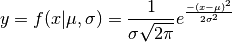The standard normal distribution has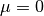and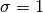.

Parameters
mufloat, optional

Normal distribution mean. Default 0.0.

sigmafloat, optional

Normal distribution standard deviation. Default 1.0.

Returns
CArray

Normal distribution values.

Examples

>>> from secml.array import CArray

>>> print(CArray([1,2,3]).normpdf())
CArray([0.241971 0.053991 0.004432])

>>> print(CArray([1,2,3]).normpdf(2,0.5))
CArray([0.107982 0.797885 0.107982])

classmethod ones(shape, dtype=<class 'float'>, sparse=False)[source]

Return a new array of given shape and type, filled with ones.

Parameters
shapeint or tuple

Shape of the new array, e.g., 2 or (2,3).

dtypestr or dtype, optional

The desired data-type for the array. Default is float.

sparsebool, optional

If False (default) a dense array will be returned. Otherwise, a sparse array of ones is created.

Returns
CArray

Array of ones with the given properties.

Warning

When sparse is True, array is created as dense and then converted to sparse format. Consequently, the performance of this method is not comparable to other sparse array creation routines.

Examples

>>> from secml.array import CArray

>>> print(CArray.ones(2))
CArray([1. 1.])

>>> print(CArray.ones((2,1), dtype=int, sparse=True))
CArray(  (0, 0) 1
(1, 0)        1)

pinv(self, rcond=1e-15)[source]

Compute the (Moore-Penrose) pseudo-inverse of a matrix.

DENSE FORMAT ONLY

Calculate the generalized inverse of a matrix using its singular-value decomposition (SVD) and including all large singular values.

Parameters
rcondfloat

Cutoff for small singular values. Singular values smaller (in modulus) than rcond * largest_singular_value (again, in modulus) are set to zero.

Returns
array_pinvCArray

The pseudo-inverse of array. Resulting array have shape (array.shape, array.shape).

Raises
LinAlgErrordense only

If array is not square or inversion fails.

Notes

The pseudo-inverse of a matrix A, denoted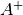, is defined as: “the matrix that ‘solves’ [the least-squares problem]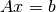,” i.e., if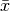is said solution, thenis that matrix such that :math:’bar{x} = A^+b’. It can be shown that if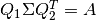is the singular value decomposition of A, then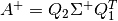, where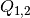are orthogonal matrices,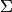is a diagonal matrix consisting of A’s so-called singular values, (followed, typically, by zeros), and then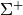is simply the diagonal matrix consisting of the reciprocals of A’s singular values (again, followed by zeros). 

References

1

G. Strang, Linear Algebra and Its Applications, 2nd Ed., Orlando, FL, Academic Press, Inc., 1980, pp. 139-142.

Examples

>>> from secml.array import CArray

The following example checks that:

array * array_pinv * array == array and array_pinv * array * array_pinv == array_pinv

>>> array = CArray([[1,3],[0,5],[8,2]])
>>> array_pinv = array.pinv()
>>> (array == array.dot(array_pinv.dot(array)).round()).all()
True

>>> (array_pinv.round(2) == array_pinv.dot(array.dot(array_pinv)).round(2)).all()
True

pow(self, exp)[source]

Array elements raised to powers from input exponent, element-wise.

Raise each base in the array to the positionally-corresponding power in exp. exp must be broadcastable to the same shape of array. If exp is a scalar, works like standard ** operator.

Parameters
expCArray or scalar

Exponent of power, can be another array (DENSE ONLY) or a single scalar. If array, must have the same shape of original array.

Returns
CArray

New array with the power of current data using input exponents.

Examples

>>> from secml.array import CArray

>>> print(CArray([1,2,3]).pow(2))
CArray([1 4 9])

>>> print(CArray([1,2,3]).pow(CArray([2,0,3])))
CArray([ 1  1 27])

>>> print(CArray([1,0,3], tosparse=True).pow(2))
CArray(  (0, 0) 1
(0, 2)        9)

prod(self, axis=None, dtype=None, keepdims=True)[source]

Return the product of array elements over a given axis.

Parameters
axisint or None, optional

Axis along which the product is computed. The default (None) is to compute the product over the flattened array.

dtypestr or dtype, optional

The data-type of the returned array, as well as of the accumulator in which the elements are multiplied. By default, if array is of integer type, dtype is the default platform integer. (Note: if the type of array is unsigned, then so is dtype.) Otherwise, the dtype is the same as that of array.

keepdimsbool, optional

If this is set to True (default), the result will broadcast correctly against the original array. Otherwise resulting array is flattened.

Returns
scalar or CArray

Product of the elements in the array. If axis is None, scalar is returned. Otherwise, a CArray with shape and number of dimensions consistent with the original array and the axis parameter is returned.

Notes

Differently from numpy, we manage flat vectors as 2-Dimensional of shape (1, array.size). This means that when axis=0, a flat array is returned as is (see examples).

Arithmetic is modular when using integer types, and no error is raised on overflow. That means that, on a 32-bit platform:

>>> print(CArray([536870910, 536870910, 536870910, 536870910]).prod())  # random result
16


Examples

>>> from secml.array import CArray

>>> print(CArray([[1,2],[3,4]]).prod())
24

>>> print(CArray([[1,2],[3,4]], tosparse=True).prod(axis=1))
CArray(  (0, 0) 2
(1, 0)        12)
>>> print(CArray([[1,2],[3,4]]).prod(axis=0, dtype=float))
CArray([[3. 8.]])

>>> print(CArray([1,2,3]).prod(axis=0))
CArray([1 2 3])
>>> print(CArray([1,2,3]).prod(axis=1))
CArray()

classmethod rand(shape, random_state=None, sparse=False, density=0.01)[source]

Return random floats in the half-open interval [0.0, 1.0).

Results are from the “continuous uniform” distribution over the stated interval. To sample Unif[a, b), b > a multiply the output of rand by (b-a) and add a:

(b - a) * rand() + a

Parameters
shapeint or tuple

Shape of the new array.

random_stateint or None, optional

If int, random_state is the seed used by the random number generator; If None, is the seed used by np.random.

sparsebool, optional

If False (default) a dense array will be returned. Otherwise, a sparse array of zeros is created.

densityscalar, optional, sparse only

Density of the generated sparse array, default 0.01 (1%). Density equal to one means a full array, density of 0 means no non-zero items.

Returns
CArray

Array of random floats with the given shape and format.

Examples

>>> from secml.array import CArray

>>> array_dense = CArray.randn(shape=2)
>>> print(array_dense)
CArray([-0.170139  0.445385])

>>> array_dense = CArray.rand(shape=(2, 3))
>>> print(array_dense)
[[ 0.68588225  0.88371576  0.3958642 ]
[ 0.58243871  0.05104796  0.77719998]]

>>> array_sparse = CArray.rand((2, 3), sparse=True, density=0.45)
>>> print(array_sparse)
CArray(  (0, 0) 0.209653887609
(1, 1)        0.521906773406)

classmethod randint(low, high=None, shape=None, random_state=None, sparse=False)[source]

Return random integers from low (inclusive) to high (exclusive).

Return random integers from the “discrete uniform” distribution in the “half-open” interval [low, high). If high is None (the default), then results are from [0, low).

Parameters
lowint

Lowest (signed) integer to be drawn from the distribution (unless high=None, in which case this parameter is the highest such integer).

highint or None, optional

If provided, one above the largest (signed) integer to be drawn from the distribution (see above for behavior if high=None).

shapeint, tuple or None, optional

Shape of output array. If None, a single value is returned.

random_stateint or None, optional

If int, random_state is the seed used by the random number generator; If None, is the seed used by np.random.

sparsebool, optional

If False (default) a dense array will be returned. Otherwise, a random sparse array is created.

Returns
CArray

Size-shaped array of random integers.

Warning

When sparse is True, array is created as dense and then converted to sparse format. Consequently, the performance of this method is not comparable to other sparse array creation routines.

Examples

>>> from secml.array import CArray

>>> array = CArray.randint(5, shape=10)
>>> print(array)
CArray([1 0 0 2 2 0 2 4 3 4])

>>> array = CArray.randint(0, 5, 10)
>>> print(array)
CArray([0 2 2 0 3 1 4 2 4 1])

>>> array = CArray.randint(0, 5, (2, 2))
>>> print(array)
CArray([[3 2]
[0 2]])

classmethod randn(shape, random_state=None)[source]

Return a sample (or samples) from the “standard normal” distribution.

DENSE FORMAT ONLY

The samples are generated from a univariate “normal” (Gaussian) distribution of mean 0 and variance 1.

Parameters
shapeint or tuple

Shape of the new array.

random_stateint or None, optional

If int, random_state is the seed used by the random number generator; If None, is the seed used by np.random.

Returns
CArray or float

A new array of given shape with floating-point samples from the standard normal distribution, or a single such float if no parameters were supplied.

Examples

>>> from secml.array import CArray

>>> array_dense = CArray.randn(shape=2)
>>> print(array_dense)
CArray([-0.739091  1.201532])

>>> array_dense = CArray.randn(shape=(2, 3))
>>> print(array_dense)
CArray([[ 0.2848132  -0.02965108  1.41184901]
[-1.3842878   0.2673215   0.18978747]])

classmethod randsample(a, shape=None, replace=False, random_state=None, sparse=False)[source]

Generates a random sample from a given array.

Parameters
aCArray or int

If an array, a random sample is generated from its elements. If an int, the random sample is generated as if a was CArray.arange(n)

shapeint, tuple or None, optional

Shape of output array. If None, a single value is returned.

replacebool, optional

Whether the sample is with or without replacement, default False.

random_stateint or None, optional

If int, random_state is the seed used by the random number generator; If None, is the seed used by np.random.

sparsebool, optional

If False (default) a dense array will be returned. Otherwise, a random sparse array is created.

Returns
CArray

The generated random samples.

Warning

When sparse is True, array is created as dense and then converted to sparse format. Consequently, the performance of this method is not comparable to other sparse array creation routines.

Examples

>>> from secml.array import CArray

>>> array = CArray.randsample(10, shape=(2, 3))
>>> print(array)
CArray([[2 9 4]
[8 6 5]])

>>> array = CArray.randsample(CArray([1,5,6,7,3]), shape=4)
>>> print(array)
CArray([3 7 5 6])

>>> CArray.randsample(3, 4)
Traceback (most recent call last):
...
ValueError: Cannot take a larger sample than population when 'replace=False'

classmethod randuniform(low=0.0, high=1.0, shape=None, random_state=None, sparse=False)[source]

Return random samples from low (inclusive) to high (exclusive).

Samples are uniformly distributed over the half-open interval [low, high) (includes low, but excludes high). In other words, any value within the given interval is equally likely to be drawn.

Parameters
lowfloat or CArray, optional
Lower boundary of the output interval. All values generated

will be greater than or equal to low. The default value is 0.

A CArray of floats can be passed to specify a different bound

for each position.

highfloat or CArray, optional
Upper boundary of the output interval. All values generated

will be less than high. The default value is 1.0.

A CArray of floats can be passed to specify a different bound

for each position.

shapeint, tuple or None, optional

Shape of output array. If None, a single value is returned.

random_stateint or None, optional
If int, random_state is the seed used by the

random number generator; If None, is the seed used by np.random.

sparsebool, optional

If False (default) a dense array will be returned. Otherwise, a random sparse array is created.

Returns
CArray

Size-shaped array of random samples.

Warning

When sparse is True, array is created as dense and then converted to sparse format. Consequently, the performance of this method is not comparable to other sparse array creation routines.

Examples

>>> from secml.array import CArray

>>> print(CArray.randuniform(high=5.0, shape=5))
CArray([ 4.36769   0.139844  3.711734  4.924484  3.737672])

>>> print(CArray.randuniform(shape=(2, 5)))
CArray([[ 0.158324  0.485235  0.723386  0.072326  0.344732]
[ 0.761642  0.844458  0.501523  0.171417  0.002068]])

>>> print(CArray.randuniform(CArray([-1, -2, 3]), 5, (2, 3)))
CArray([[ -0.584032  1.433291  3.671319]
[ 3.566163 -1.139602  4.268376]])

ravel(self)[source]

Return a flattened array.

For dense format a 1-D array, containing the elements of the input, is returned. For sparse format a (1 x array.size) array will be returned.

A copy is made only if needed.

Returns
CArray

Flattened view (if possible) of the array with shape (array.size,) for dense format or (1, array.size) for sparse format.

Examples

>>> from secml.array import CArray

>>> print(CArray([[1,2],[3,4]]).ravel())
CArray([1 2 3 4])

>>> print(CArray([,,], tosparse=True).ravel())
CArray(  (0, 0) 1
(0, 1)        2
(0, 2)        3)

repeat(self, repeats, axis=None)[source]

Repeat elements of an array.

DENSE FORMAT ONLY

Parameters
repeatsint, list or CArray

The number of repetitions for each element. If this is an array_like object, will be broadcasted to fit the shape of the given axis.

axisint, optional

The axis along which to repeat values. By default, array is flattened before use.

Returns
CArray

Output array which has the same shape as original array, except along the given axis. If axis is None, a flat array is returned.

Examples

>>> from secml.array import CArray

>>> x = CArray([[1,2],[3,4]])

>>> print(x.repeat(2))
CArray([1 1 2 2 3 3 4 4])

>>> print(x.repeat(2, axis=1))  # Repeat the columns on the right
CArray([[1 1 2 2]
[3 3 4 4]])
>>> print(x.repeat(2, axis=0))  # Repeat the rows on the right
CArray([[1 2]
[1 2]
[3 4]
[3 4]])

>>> print(x.repeat([1, 2], axis=0))
CArray([[1 2]
[3 4]
[3 4]])

>>> x.repeat([1, 2])  # repeats size must be consistent with axis
Traceback (most recent call last):
...
ValueError: operands could not be broadcast together with shape (4,) (2,)

>>> x = CArray([1,2,3])
>>> print(x.repeat(2, axis=0))  # Repeat the (only) row on the right
CArray([1 1 2 2 3 3])
>>> print(x.repeat(2, axis=1))  # No columns to repeat
Traceback (most recent call last):
...
numpy.core._internal.AxisError: axis 1 is out of bounds for array of dimension 1

repmat(self, m, n)[source]

Repeat an array M x N times.

Parameters
m, nint

The number of times the array is repeated along the first and second axes.

Returns
CArray

The result of repeating array m X n times.

Examples

>>> from secml.array import CArray

>>> print(CArray([[1,2]],tosparse=True).repmat(2,2))
CArray(  (0, 0) 1
(0, 1)        2
(0, 2)        1
(0, 3)        2
(1, 0)        1
(1, 1)        2
(1, 2)        1
(1, 3)        2)

>>> print(CArray([1,2]).repmat(2,2))
CArray([[1 2 1 2]
[1 2 1 2]])
>>> print(CArray([1,2]).repmat(1,2))
CArray([[1 2 1 2]])
>>> print(CArray([1,2]).repmat(2,1))
CArray([[1 2]
[1 2]])

reshape(self, newshape)[source]

Gives a new shape to an array without changing its data.

Parameters
newshapeint or sequence of ints

Desired shape for output array. If an integer or a tuple of length 1, resulting array will have shape (n,) if dense, (1,n) if sparse.

A copy is made only if needed.
Returns
CArray

Array with new shape. If possible, a view of original array data will be returned, otherwise a copy will be made first.

Examples

>>> from secml.array import CArray

>>> print(CArray([1,2,3]).reshape((3,1)))
CArray([

])

>>> print(CArray([,,], tosparse=True).reshape(3))
CArray(  (0, 0) 1
(0, 1)        2
(0, 2)        3)

>>> CArray([1,2,3]).reshape(4)
Traceback (most recent call last):
...
ValueError: cannot reshape array of size 3 into shape (4,)

resize(self, newshape, constant=0)[source]

Return a new array with the specified shape.

Missing entries are filled with input constant (default 0).

DENSE FORMAT ONLY

Parameters
newshapeint or sequence of ints

Integer or one integer for each desired dimension of output array. If a tuple of length 1, output sparse array will have shape (1, n).

constantscalar

Scalar to be used for filling missing entries. Default 0.

Returns
CArray

Array with new shape. Array dtype is preserved. Missing entries are filled with the desired constant (default 0).

Examples

>>> from secml.array import CArray

>>> print(CArray([1,2,3]).resize((3,3)))
CArray([[1 2 3]
[0 0 0]
[0 0 0]])

>>> print(CArray([1,2,3]).resize((3,1)))
CArray([

])

>>> print(CArray([1,2,3]).resize((1,3)))
CArray([[1 2 3]])

>>> print(CArray([[1,2,3]]).resize((5, )))
CArray([1 2 3 0 0])

>>> from secml.core.constants import inf
>>> print(CArray([[1,2,3]]).resize((5, ), constant=inf))
CArray([                   1                    2                    3
-9223372036854775808 -9223372036854775808])

>>> print(CArray([[0, 1],[2, 3]]).resize(3))
CArray([0 1 2])

>>> print(CArray([[0, 1],[2, 3]]).resize((3, 3)))
CArray([[0 1 2]
[3 0 0]
[0 0 0]])

>>> print(CArray([[0, 1, 2],[3, 4, 5]]).resize((2, 2)))
CArray([[0 1]
[2 3]])

round(self, decimals=0)[source]

Evenly round to the given number of decimals.

Parameters
decimalsint, optional

Number of decimal places to round to (default: 0). If decimals is negative, it specifies the number of positions to round to the left of the decimal point.

Returns
out_roundedCArray

An new array containing the rounded values. The real and imaginary parts of complex numbers are rounded separately. The result of rounding a float is a float.

ceil

Return the ceiling of the input, element-wise.

floor

Return the floor of the input, element-wise.

Notes

For values exactly halfway between rounded decimal values, we rounds to the nearest even value. Thus 1.5 and 2.5 round to 2.0, -0.5 and 0.5 round to 0.0, etc. Results may also be surprising due to the inexact representation of decimal fractions in the IEEE floating point standard  and errors introduced when scaling by powers of ten.

References

1

“Lecture Notes on the Status of IEEE 754”, William Kahan, http://www.cs.berkeley.edu/~wkahan/ieee754status/IEEE754.PDF

2

“How Futile are Mindless Assessments of Roundoff in Floating-Point Computation?”, William Kahan, http://www.cs.berkeley.edu/~wkahan/Mindless.pdf

Examples

>>> from secml.array import CArray

>>> print(CArray([1.28,5.62]).round())
CArray([1. 6.])

>>> print(CArray([1.28,5.62],tosparse=True).round(decimals=1))
CArray(  (0, 0) 1.3
(0, 1)        5.6)

>>> print(CArray([.5, 1.5, 2.5, 3.5, 4.5]).round()) # rounds to nearest even value
CArray([0. 2. 2. 4. 4.])

>>> print(CArray([1,5,6,11]).round(decimals=-1))
CArray([ 0  0 10 10])

save(self, datafile, overwrite=False)[source]

Save array data into plain text file.

Data is stored preserving original data type.

The default encoding is utf-8.

Parameters
datafilestr, file_handle (dense only)

Text file to save data to. If a string, it’s supposed to be the filename of file to save. If a file handle, data will be stored using active file handle mode. If the filename ends in .gz, the file is automatically saved in compressed gzip format. load() function understands gzipped files transparently.

overwritebool, optional

If True and target file already exists, file will be overwritten. Otherwise (default), IOError will be raised.

Notes

• Dense format, flat arrays are stored with shape N x 1.

• Sparse format, we only save non-zero data along with indices

necessary to reconstruct original 2-dimensional array.

• Dense format, shape of original array can be easily recognized

from target text file.

sha1(self)[source]

Calculate the sha1 hexadecimal hash of array.

Returns
hashstr

Examples

>>> from secml.array import CArray

>>> print(CArray([0,1,3]).sha1())
9d9d15176c022373488fb8a2b34be0ba3046f5c6

property shape

Shape of stored data, tuple of ints.

shuffle(self)[source]

Modify array in-place by shuffling its contents.

This function only shuffles the array along the first index of a not vector-like, multi-dimensional array.

Examples

>>> from secml.array import CArray

>>> array = CArray([2,3,0,1])
>>> array.shuffle()
>>> print(array)
CArray([0 2 1 3])  # random result

>>> array = CArray([[2,3],[0,1]])
>>> array.shuffle()
>>> print(array)
CArray([[0 1]
[2 3]])

sign(self)[source]

Returns element-wise sign of the array.

The sign function returns -1 if x < 0, 0 if x == 0, 1 if x > 0.

Returns
CArray

Array with sign of each element.

Examples

>>> from secml.array import CArray

>>> print(CArray([[-2,0,2]]).sign())
CArray([[-1  0  1]])

>>> print(CArray([-2,0,2], tosparse=True).sign())
CArray(  (0, 0) -1
(0, 2)        1)

sin(self)[source]

Trigonometric sine, element-wise.

DENSE FORMAT ONLY

The array elements are considered angles, in radians (rad equals 360 degrees).

Returns
CArray

New array with trigonometric sine element-wise.

Notes

The sine is one of the fundamental functions of trigonometry (the mathematical study of triangles). Consider a circle of radius 1 centered on the origin. A ray comes in from the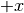axis, makes an angle at the origin (measured counter-clockwise from that axis), and departs from the origin. The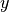coordinate of the outgoing ray’s intersection with the unit circle is the sine of that angle. It ranges from -1 for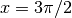to +1 for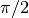. The function has zeroes where the angle is a multiple of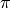. Sines of angles betweenandare negative. The numerous properties of the sine and related functions are included in any standard trigonometry text.

Examples

>>> from secml.array import CArray
>>> from secml.core.constants import pi

>>> print((CArray([0,90,180,270,360,-90,-180,-270])*pi/180).sin().round())
CArray([ 0.  1.  0. -1. -0. -1. -0.  1.])

>>> print((CArray([[45,135],[225,315]])*pi/180).sin())
CArray([[ 0.707107  0.707107]
[-0.707107 -0.707107]])

property size

Size (number of elements) of array.

For sparse data, this counts both zeros and non-zero elements.

sort(self, axis=-1, kind='quicksort', inplace=False)[source]

Sort an array.

Parameters
axisint, optional

Axis along which to sort. The default is -1 (the last axis).

kind{‘quicksort’, ‘mergesort’, ‘heapsort’}, optional

Sorting algorithm to use. Default ‘quicksort’. For sparse arrays, only ‘quicksort’ is available.

inplacebool, optional

If True, array will be sorted in-place. Default False.

Returns
CArray

Sorted array.

numpy.sort

Description of different sorting algorithms.

CArray.argsort

Indirect sort.

Notes

Differently from numpy, we manage flat vectors as 2-Dimensional of shape (1, array.size). This means that when axis=0, flat array is returned as is (see examples).

For large sparse arrays is actually faster to convert to dense first.

Examples

>>> from secml.array import CArray

>>> array = CArray([5,-1,0,-3])
>>> print(array.sort())
CArray([-3 -1  0  5])

>>> array = CArray([5,-1,0,-3])
>>> print(array.sort(axis=0))
CArray([ 5 -1  0 -3])

>>> array = CArray([5,-1,0,-3])
>>> print(array.sort(axis=1))
CArray([-3 -1  0  5])

>>> array = CArray([5,-1,0,-3])
>>> out = array.sort(inplace=True)
>>> print(out)
CArray([-3 -1  0  5])
>>> array = 100
>>> print(out)
CArray([100  -1   0   5])

sqrt(self)[source]

Compute the positive square-root of an array, element-wise.

If any array element is complex, a complex array is returned (and the square-roots of negative reals are calculated). If all of the array elements are real, so is the resulting array, with negative elements returning nan.

Returns
CArray

A new array with the element-wise positive square-root of original array.

Notes

sqrt has, consistent with common convention, its branch cut the real “interval” [-inf, 0), and is continuous from above on it. A branch cut is a curve in the complex plane across which a given complex function fails to be continuous.

Examples

>>> from secml.array import CArray

>>> print(CArray(2).sqrt())
CArray([1.414214])

>>> print(CArray([2,3,4]).sqrt())
CArray([1.414214 1.732051 2.      ])

>>> print(CArray([[2,3],[4,5]],tosparse=True).sqrt().round(4))
CArray(  (0, 0) 1.4142
(0, 1)        1.7321
(1, 0)        2.0
(1, 1)        2.2361)

>>> print(CArray([-3, 0]).sqrt())
CArray([nan  0.])

std(self, axis=None, ddof=0, keepdims=True)[source]

Compute the standard deviation along the specified axis.

Returns the standard deviation, a measure of the spread of a distribution, of the array elements. The standard deviation is computed for the flattened array by default, otherwise over the specified axis.

Parameters
axisint, optional

Axis along which the standard deviation is computed. The default is to compute the standard deviation of the flattened array.

ddofint, optional

Means Delta Degrees of Freedom. The divisor used in calculations is N - ddof, where N represents the number of elements. By default ddof is zero.

keepdimsbool, optional

If this is set to True (default), the result will broadcast correctly against the original array.

Returns
float or CArray

Standard deviation of the elements in the array. If axis is None, float is returned. Otherwise, a CArray with shape and number of dimensions consistent with the original array and the axis parameter is returned.

Notes

The standard deviation is the square root of the average of the squared deviations from the mean, i.e., ‘std = sqrt(mean(abs(x - x.mean())**2))’.

The average squared deviation is normally calculated as ‘x.sum() / N’, where ‘N = len(x)’. If, however, ddof is specified, the divisor ‘N - ddof’ is used instead. In standard statistical practice, ‘ddof=1’ provides an unbiased estimator of the variance of the infinite population. ‘ddof=0’ provides a maximum likelihood estimate of the variance for normally distributed variables. The standard deviation computed in this function is the square root of the estimated variance, so even with ‘ddof=1’, it will not be an unbiased estimate of the standard deviation per se.

Note that, for complex numbers, std takes the absolute value before squaring, so that the result is always real and not-negative.

For floating-point input, the mean is computed using default float precision. Depending on the input data, this can cause the results to be inaccurate, especially for 32-bit machines (float32).

Examples

>>> from secml.array import CArray

>>> print(round(CArray([[1,4],[4,3]],tosparse=True).std(), 2))
1.22

>>> print(CArray([[1,4],[4,3]],tosparse=True).std(axis=0))
CArray([[1.5 0.5]])

>>> print(CArray([[1,4],[4,3]]).std(axis=0, ddof=1).round(2))
CArray([[2.12 0.71]])

>>> print(CArray([1,4,4,3]).std(axis=0))
CArray([0. 0. 0. 0.])
>>> print(CArray([1,4,4,3]).std(axis=1).round(2))
CArray([1.22])

sum(self, axis=None, keepdims=True)[source]

Sum of array elements over a given axis.

Parameters
axisint or None, optional

Axis along which a sum is performed. The default (axis = None) is perform a sum over all the dimensions of the input array. axis may be negative, in which case it counts from the last to the first axis.

keepdimsbool, optional

If this is set to True (default), the result will broadcast correctly against the original array. Otherwise resulting array is flattened.

Returns
scalar or CArray

Sum of the elements in the array. If axis is None, float is returned. Otherwise, a CArray with shape and number of dimensions consistent with the original array and the axis parameter is returned.

Examples

>>> from secml.array import CArray

>>> print(CArray([-3,0,2]).sum())
-1

>>> print(CArray([[-3,0],[1,2]], tosparse=True).sum(axis=1))
CArray([[-3]
[ 3]])

>>> print(CArray([-3,0,1,2]).sum(axis=0))
CArray([-3  0  1  2])
>>> print(CArray([-3,0,1,2]).sum(axis=1))
CArray()

t
tocsr(self)[source]

Return a sparse scipy.sparse.csr_matrix representation of array.

Returns
scipy.sparse.csr_matrix

A representation of current data as scipy.sparse.csr_matrix. If possible, we avoid copying original data.

Examples

>>> from secml.array import CArray

>>> array = CArray([[1,2],[0,4]], tosparse=True).tocsr()
>>> print(array)
(0, 0)        1
(0, 1)        2
(1, 1)        4
>>> type(array)
<class 'scipy.sparse.csr.csr_matrix'>

>>> array = CArray([1,2,3]).tocsr()
>>> print(array)
(0, 0)        1
(0, 1)        2
(0, 2)        3
>>> type(array)
<class 'scipy.sparse.csr.csr_matrix'>

todense(self, dtype=None, shape=None)[source]

Converts array to dense format.

Return current array if it has already a dense format.

Parameters
dtypestr or dtype, optional

Typecode or data-type to which the array is cast.

shapesequence of ints, optional

Shape of the new array, e.g., ‘(2, 3)’.

Returns
CArray

Dense array with input data and desired dtype and/or shape.

Notes

If current array has already a dense format, dtype and shape parameters will not be functional. Use .astype() or .reshape() function to alter array shape/dtype.

Examples

>>> from secml.array import CArray

>>> print(CArray([[2, 0], [3, 4]], tosparse=True).todense(dtype=float))
CArray([[2. 0.]
[3. 4.]])

>>> print(CArray([[2, 0], [3, 4]], tosparse=True).todense(shape=(4,)))
CArray([2 0 3 4])

tolist(self)[source]

Return the array as a (possibly nested) list.

Return a copy of the array data as a (nested) Python list. Data items are converted to the nearest compatible Python type.

Returns
list

The possibly nested list of array elements.

Examples

>>> from secml.array import CArray

>>> array = CArray([[1,2],[0,4]]).tolist()
>>> array
[[1, 2], [0, 4]]
>>> print(CArray(array))
CArray([[1 2]
[0 4]])

>>> print(CArray(array, tosparse=True))
CArray(  (0, 0) 1
(0, 1)        2
(1, 1)        4)

tondarray(self)[source]

Return a dense numpy.ndarray representation of array.

Returns
numpy.ndarray

A representation of current data as numpy.ndarray. If possible, we avoid copying original data.

Examples

>>> from secml.array import CArray

>>> array = CArray([1,2,3]).tondarray()
>>> array
array([1, 2, 3])
>>> type(array)
<class 'numpy.ndarray'>

>>> array = CArray([[1,2],[0,4]],tosparse=True).tondarray()
>>> array
array([[1, 2],
[0, 4]], dtype=int64)
>>> type(array)
<class 'numpy.ndarray'>

tosparse(self, dtype=None, shape=None)[source]

Converts array to sparse format.

Return current array if it has already a sparse format.

Parameters
dtypestr or dtype, optional

Typecode or data-type to which the array is cast.

shapesequence of ints, optional

Shape of the new array, e.g., ‘(2, 3)’. Only 2-Dimensional sparse arrays are supported.

Returns
CArray

Sparse array with input data and desired dtype and/or shape.

Notes

If current array has already a sparse format, dtype and shape parameters will not be functional. Use .astype() or .reshape() function to alter array shape/dtype.

Examples

>>> from secml.array import CArray

>>> print(CArray([[2, 0], [3, 4]]).tosparse(dtype=float))
CArray(  (0, 0) 2.0
(1, 0)        3.0
(1, 1)        4.0)

>>> print(CArray([[2, 0], [3, 4]]).tosparse(shape=(1, 4)))
CArray(  (0, 0) 2
(0, 2)        3
(0, 3)        4)

transpose(self)[source]

Returns current array with axes transposed.

A view is returned if possible.

Returns
CArray

A view, if possible, of current array with axes suitably permuted.

Examples

>>> from secml.array import CArray

>>> print(CArray([1, 2, 3]).transpose())
CArray([

])

>>> print(CArray([, , ]).transpose())
CArray([[1 2 3]])

unique(self, return_index=False, return_inverse=False, return_counts=False)[source]

Find the unique elements of an array.

There are three optional outputs in addition to the unique elements: - the indices of the input array that give the unique values - the indices of the unique array that reconstruct the input array - the number of times each unique value comes up in the input array

Parameters
return_indexbool, optional

If True, also return the indices of array that result in the unique array (default False).

return_inversebool, optional, dense only

If True, also return the indices of the unique array that can be used to reconstruct the original array (default False).

return_countsbool, optional

If True, also return the number of times each unique item appears.

Returns
uniqueCArray

Dense array with the sorted unique values of the array.

unique_indexCArray, optional

The indices of the first occurrences of the unique values in the (flattened) original array. Only provided if return_index is True.

unique_countsCArray, optional

The number of times each unique item appears in the original array. Only provided if return_counts is True.

Examples

>>> from secml.array import CArray

>>> print(CArray([[1,0,2],[2,0,3]]).unique())
CArray([0 1 2 3])

>>> print(CArray([1,2,2,3,3], tosparse=True).unique())
CArray([1 2 3])

>>> u, u_idx, u_inv = CArray([[2,2,3,3]]).unique(return_index=True, return_inverse=True)
>>> print(u)  # unique
CArray([2 3])
>>> print(u_idx)  # unique_indices
CArray([0 2])
>>> print(u[u_inv])  # original (flattened) array reconstructed from unique_inverse
CArray([2 2 3 3])

>>> u, u_counts = CArray([[2,2,2,3,3]]).unique(return_counts=True)
>>> print(u_counts)  # The number of times each unique item appears
CArray([3 2])

classmethod zeros(shape, dtype=<class 'float'>, sparse=False)[source]

Return a new array of given shape and type, filled with zeros.

Parameters
shapeint or tuple

Shape of the new array, e.g., 2 or (2,3).

dtypestr or dtype, optional

The desired data-type for the array. Default is float.

sparsebool, optional

If False (default) a dense array will be returned. Otherwise, a sparse array of zeros is created. Note that sparse arrays with only zeros appear empty when printing.

Returns
CArray

Array of zeros with the given properties.

Examples

>>> from secml.array import CArray

>>> print(CArray.zeros(2))
CArray([0. 0.])

>>> array = CArray.zeros((2,1), dtype=int, sparse=True)
>>> print(array)  # sparse arrays with only zeros appear empty...
CArray()
>>> print(array.shape)
(2, 1)


## array_utils¶

secml.array.array_utils.is_vector_index(idx)[source]

Check if input index is valid for vector-like arrays.

An array is vector-like when 1-Dimensional or 2-Dimensional with shape == 1.

Parameters
idxint, bool, slice

Index to check.

Returns
out_checkbool

Return True if input is a valid index for any axis with size 1, else False.

secml.array.array_utils.tuple_atomic_tolist(idx)[source]

Convert tuple atomic elements to list.

Atomic objects converted:
• int, np.integer

• bool, np.bool_

Parameters
idxtuple

Tuple which elements have to be converted.

Returns
out_tupletuple

Converted tuple.

secml.array.array_utils.tuple_sequence_tondarray(idx)[source]

Convert sequences inside tuple to ndarray.

A sequence can be:
• int, np.integer

• bool, np.bool_

• list

• np.ndarray

• CDense

• CSparse (are converted to dense first)

• CArray

Parameters
idxtuple

Tuple which elements have to be converted.

Returns
out_tupletuple

Converted tuple.